Complex Klein-Gordon field: dead ends take III (or more?)

September 29, 2018 phy2403 2 comments

Working on a problem from Peskin and Schroeder (and Zee too actually), I’ve hit dead ends in many different ways. The last dead end looks impressive just sitting there on the table where I abandoned it: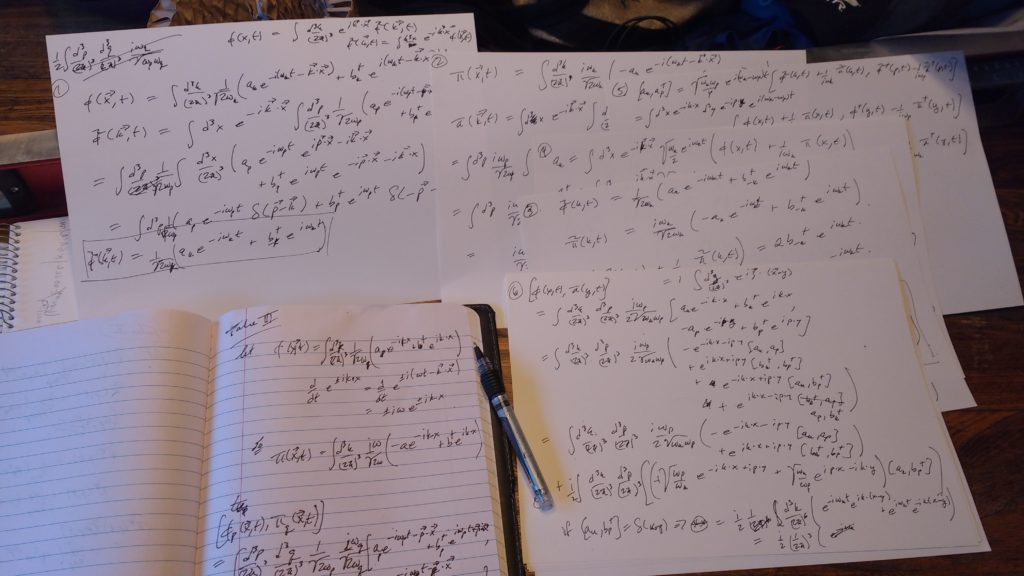… however, after getting results that weren’t expected a number of different ways, I think I finally understand the fundamental assumption that I had wrong.  Take >= IV.

The identity ideologues are pushing hard from york region primary schools

York region district school board is preparing to roll out their “Every Student Counts Survey” for primary grades (to be answered with parental assistance) or by oneself in grade 7-12 that’s packed full of the extreme identity politics that is discussed so widely in podcasts these days.

Here’s a link to a sample copy of the survey for kindergarden to grade 6, and one for higher grades.  The one for the little kids is particularly absurd, asking the kids or parents to pick from gender identities of male, female or one of the following 7 additional options:

• Gender Fluid (Of, relating to, or being a person whose gender identity or expression changes or shifts along thegender spectrum.)
• Gender Nonconforming (Not being in line with the cultural associations made in a given society about a person’s
sex assigned at birth.)
• Non‐Binary (Refers to a person whose gender identity does not align with the binary concept of gender such as
man or woman.)
• Questioning (Refers to a person who is unsure about their own gender identity.)
• Transgender (Refers to a person whose gender identity differs from the one associated with their birth‐assigned
sex.)
• Two‐Spirit (An Indigenous person whose gender identity, spiritual identity or sexual orientation includes
masculine, feminine or non‐binary spirits.)
• A gender identity not listed above (please specify):…

as constrained by the footnote: “A person’s internal and deeply felt sense of being a man, a woman, both, neither, or having another identity on the gender spectrum. A person’s gender identity may be different from the sex assigned at birth (for example, female, intersex, male).”

These are questions being asked of kids that are potentially still many years away from puberty!  Why on earth is there such pressure to have the kids pick from or even try to understand these host of made up categories?

When I was in grade 7 I got teased about being gay because I was too shy to ask a girl on a date (or accept a date, in one instance).  Heck, I was a virgin until I was in my early twenties.  If this level of gender propaganda was being pushed when I was a kid, I’d have to have started wearing a lipstick and blouses just to fit it.  All because I was introverted and shy.  My sexual identity had nothing to do with these plethora of current gender categories, but just because I hadn’t clued in that you had to communicate to the opposite sex if you wanted to make any progress towards sexual goals.  Time is required to figure this stuff out, and having to choose prematurely, seems, to be blunt, completely stupid.

There’s some other stuff in this survey that is just bizarre.  The desire to label is so severe that it appears they are also making up new races:

Latino/Latina/Latinx

Googling Latinx, which I hadn’t heard of, and isn’t defined in any of the “helpful” footnotes, it appears that they are pushing gender politics into race too.  Wikipedia says of this: “Latinx (la-teen-ex) is a gender-neutral term sometimes used in lieu of Latino or Latina”.

Here’s some samples of the gobbledegook that this survey is packed full of:

• People can be treated differently based on their religion, or perceived religion, which can lead to negative impacts and unequal outcomes. Islamophobia and antisemitism are examples of the way religion can be racialized. People can experience racism not only based on skin colour but also other perceived characteristics that are associated with religion.
• Bullying is an ongoing misuse of power in relationships through repeated verbal, physical and/or social behaviour that causes physical and/or psychological harm. It can involve an individual or a group misusing their power over one or more persons. Bullying can happen in person or online, and it can be obvious (overt) or hidden (covert).
• Discrimination is being treated negatively because of your gender, racial background, ethnic origin, religion, socioeconomic background, special education needs, sexual orientation, or other factors. Discrimination can be intentional or unintentional.
• Harassment is engaging in a course of vexatious [annoying or provoking] comment or conduct which is known or
ought reasonably to be known to be unwelcome.
• Race is a social construct that groups people on the basis of perceived common ancestry and characteristics and affects how some people are perceived and treated. Race is often confused with ethnicity (a group of people who share a particular cultural heritage or background); there may be several ethnic groups within a racialized group.

I wonder how much money York region is paying to roll out and process this survey?  As well as injecting chaos and insanity into the school system in liew of actual content, it seems like a pointless waste of time and money that will provide little useful information.

If you want to make progress in education, how about stripping out some of the crap.  There’s no shortage of that.  Let’s not confuse kids with four different pictoral multiplication algorithms, so that they’ll give up and end up using calculators.  How about not injecting terminology into math education like “commutative” in grade 5 when you won’t see non-commutative (i.e. matrix) multiplication until university (since linear algebra seems to have been dropped from the high school curriculum for all intents and purposes).  Arg!

PHY2403H Quantum Field Theory. Lecture 6: Canonical quantization, Simple Harmonic Oscillators, Symmetries. Taught by Prof. Erich Poppitz

DISCLAIMER: Very rough notes from class, with some additional side notes (QM SHO review, …).

These are notes for the UofT course PHY2403H, Quantum Field Theory I, taught by Prof. Erich Poppitz fall 2018.

Quantization of Field Theory

We are engaging in the “canonical” or Hamiltonian method of quantization. It is also possible to quantize using path integrals, but it is hard to prove that operators are unitary doing so. In fact, the mechanism used to show unitarity from path integrals is often to find the Lagrangian and show that there is a Hilbert space (i.e. using canonical quantization). Canonical quantization essentially demands that the fields obey a commutator relation of the following form
\begin{equation}\label{eqn:qftLecture6:20}
\antisymmetric{\pi(\Bx, t)}{\phi(\By, t)} = -i \delta^3(\Bx – \By).
\end{equation}
We assumed that the quantized fields obey the Hamiltonian relations
\begin{equation}\label{eqn:qftLecture6:160}
\begin{aligned}
\ddt{\phi} &= i \antisymmetric{H}{\phi} \\
\ddt{\pi} &= i \antisymmetric{H}{\pi}.
\end{aligned}
\end{equation}

We were working with the Hamiltonian density
\begin{equation}\label{eqn:qftLecture6:40}
\mathcal{H} =
\inv{2} (\pi(\Bx, t))^2
+
+
\frac{m^2}{2} \phi^2
+
\frac{\lambda}{4} \phi^4,
\end{equation}
which included a mass term $$m$$ and a potential term ($$\lambda$$). We will expand all quantities in Taylor series in $$\lambda$$ assuming they have a structure such as
\begin{equation}\label{eqn:qftLecture6:180}
\begin{aligned}
f(\lambda) =
c_0 \lambda^0
+ c_1 \lambda^1
+ c_2 \lambda^2
+ c_3 \lambda^3
+ \cdots
\end{aligned}
\end{equation}
We will stop this \underline{perturbation theory} approach at $$O(\lambda^2)$$, and will ignore functions such as $$e^{-1/\lambda}$$.

Within perturbation theory, to leaving order, set $$\lambda = 0$$, so that $$\phi$$ obeys the Klein-Gordon equation (if $$m = 0$$ we have just a d’Lambertian (wave equation)).

We can write our field as a Fourier transform
\begin{equation}\label{eqn:qftLecture6:60}
\phi(\Bx, t) = \int \frac{d^3 p}{(2\pi)^3} e^{i \Bp \cdot \Bx} \tilde{\phi}(\Bp, t),
\end{equation}
and due to a Hermitian assumption (i.e. real field) this implies
\begin{equation}\label{eqn:qftLecture6:80}
\tilde{\phi}^\conj(\Bp, t) = \tilde{\phi}(-\Bp, t).
\end{equation}

We found that the Klein-Gordon equation implied that the momentum space representation obey Harmonic oscillator equations
\begin{equation}\label{eqn:qftLecture6:100}
\begin{aligned}
\ddot{\tilde{\phi}}(\Bp, t) &= – \omega_\Bp \tilde{\phi}(\Bp, t) \\
\omega_\Bp &= \sqrt{\Bp^2 + m^2}.
\end{aligned}
\end{equation}
We may represent the solution to this equation as
\begin{equation}\label{eqn:qftLecture6:120}
\tilde{\phi}(\Bq, t) = \inv{\sqrt{2 \omega_\Bq}} \lr{
e^{-i \omega_\Bq t} a_\Bq
+
e^{i \omega_\Bq t} b_\Bq^\conj
}.
\end{equation}
This is a general solution, but imposing $$a_\Bq = b_{-\Bq}$$ ensures \ref{eqn:qftLecture6:80} is satisfied.
This leaves us with
\begin{equation}\label{eqn:qftLecture6:140}
\tilde{\phi}(\Bq, t) = \inv{\sqrt{2 \omega_\Bq}} \lr{
e^{-i \omega_\Bq t} a_\Bq
+
e^{i \omega_\Bq t} a_{-\Bq}^\conj
}.
\end{equation}
We want to show that iff
\begin{equation}\label{eqn:qftLecture6:200}
\antisymmetric{a_\Bq}{a^\dagger_\Bp} = \lr{ 2 \pi }^3 \delta^3(\Bp – \Bq),
\end{equation}
then
\begin{equation}\label{eqn:qftLecture6:220}
\antisymmetric{\pi(\By, t)}{\phi(\Bx, t)} = -i \delta^3(\Bx – \By)
\end{equation}
where everything else commutes (i.e. $$\antisymmetric{a_\Bp}{a_\Bq} = \antisymmetric{a_\Bp^\dagger}{a_\Bq^\dagger} = 0$$).
We will only show one direction, but you can go the other way too.

\begin{equation}\label{eqn:qftLecture6:240}
\phi(\Bx, t)
=
\int \frac{d^3 p}{(2\pi)^3 \sqrt{2 \omega_\Bp}} e^{i \Bp \cdot \Bx}
\lr{
e^{-i \omega_\Bp t} a_\Bp
+
e^{i \omega_\Bp t} a_{-\Bp}^\dagger
}
\end{equation}
\begin{equation}\label{eqn:qftLecture6:260}
\pi(\Bx, t) = \dot{\phi}
=
i \int \frac{d^3 q}{(2\pi)^3 \sqrt{2 \omega_\Bq}} \omega_\Bq e^{i \Bq \cdot \Bx}
\lr{
-e^{-i \omega_\Bq t} a_\Bq
+
e^{i \omega_\Bq t} a_{-\Bq}^\dagger
}
\end{equation}
The commutator is
\begin{equation}\label{eqn:qftLecture6:280}
\begin{aligned}
\antisymmetric{\pi(\By, t)}{\phi(\Bx, t)}
&=
i \int \frac{d^3 p}{(2\pi)^3
\sqrt{2 \omega_\Bp}}
\frac{d^3 q}{(2\pi)^3 \sqrt{2 \omega_\Bq}}
\omega_\Bq
e^{i \Bp \cdot \By + i \Bq \cdot \Bx}
\antisymmetric
{
-e^{-i \omega_\Bq t} a_\Bq
+
e^{i \omega_\Bq t} a_{-\Bq}^\dagger
}
{
e^{-i \omega_\Bp t} a_\Bp
+
e^{i \omega_\Bp t} a_{-\Bp}^\dagger
} \\
&=
i \int \frac{d^3 p}{(2\pi)^3
\sqrt{2 \omega_\Bp}}
\frac{d^3 q}{(2\pi)^3 \sqrt{2 \omega_\Bq}}
\omega_\Bq
e^{i \Bp \cdot \By + i \Bq \cdot \Bx}
\lr{
– e^{i (\omega_\Bp -\omega_\Bq) t}
\antisymmetric { a_\Bq } { a_{-\Bp}^\dagger }
+
e^{i (\omega_\Bq -\omega_\Bp) t}
\antisymmetric{a_{-\Bq}^\dagger}{ a_\Bp }
} \\
&=
i \int \frac{d^3 p}{(2\pi)^3
\sqrt{2 \omega_\Bp}}
\frac{d^3 q}{(2\pi)^3 \sqrt{2 \omega_\Bq}}
\omega_\Bq (2\pi)^3
e^{i \Bp \cdot \By + i \Bq \cdot \Bx}
\lr{
– e^{i (\omega_\Bp -\omega_\Bq) t} \delta^3(\Bq + \Bp)
– e^{i (\omega_\Bq -\omega_\Bp) t} \delta^3(-\Bq -\Bp)
} \\
&=
– 2 i \int \frac{d^3 p}{(2\pi)^3
2 \omega_\Bp}
\omega_\Bp
e^{i \Bp \cdot (\By – \Bx)} \\
&=
-i \delta^3(\By – \Bx),
\end{aligned}
\end{equation}
which is what we wanted to prove.

Free Hamiltonian

We call the $$\lambda = 0$$ case the “free” Hamiltonian.

\begin{equation}\label{eqn:qftLecture6:300}
\begin{aligned}
H
&= \int d^3 x \lr{ \inv{2} \pi^2 + \inv{2} (\spacegrad \phi)^2 + \frac{m^2}{2} \phi^2 } \\
&=
\inv{2} \int d^3 x
\frac{d^3 p}{(2\pi)^3}
\frac{d^3 q}{(2\pi)^3}
\frac{
e^{i (\Bp + \Bq)\cdot \Bx}
}{\sqrt{2 \omega_\Bp}
\sqrt{2 \omega_\Bq}}
\lr{

(\omega_\Bp)
(\omega_\Bq)
\lr{
-e^{-i \omega_\Bp t} a_\Bp
+
e^{i \omega_\Bp t} a_{-\Bp}^\dagger
}
\lr{
-e^{-i \omega_\Bq t} a_\Bq
+
e^{i \omega_\Bq t} a_{-\Bq}^\dagger
}
}

\Bp \cdot \Bq
\lr{
e^{-i \omega_\Bp t} a_\Bp
+
e^{i \omega_\Bp t} a_{-\Bp}^\dagger
}
\lr{
e^{-i \omega_\Bq t} a_\Bq
+
e^{i \omega_\Bq t} a_{-\Bq}^\dagger
}
+
m^2
\lr{
e^{-i \omega_\Bp t} a_\Bp
+
e^{i \omega_\Bp t} a_{-\Bp}^\dagger
}
\lr{
e^{-i \omega_\Bq t} a_\Bq
+
e^{i \omega_\Bq t} a_{-\Bq}^\dagger
}.
\end{aligned}
\end{equation}
An immediate simplification is possible by identifying a delta function factor $$\int d^3 x e^{i(\Bp + \Bq) \cdot \Bx}/(2\pi)^3 = \delta^3(\Bp + \Bq)$$, so
\begin{equation}\label{eqn:qftLecture6:1060}
\begin{aligned}
H
&=
\inv{2}
\int
\frac{d^3 p}{(2\pi)^3}
\frac{1
}{2 \omega_\Bp}
\lr{

(\omega_\Bp)^2
\lr{
-e^{-i \omega_\Bp t} a_\Bp
+
e^{i \omega_\Bp t} a_{-\Bp}^\dagger
}
\lr{
-e^{-i \omega_\Bp t} a_{-\Bp}
+
e^{i \omega_\Bp t} a_{\Bp}^\dagger
}
}
+
(\Bp^2 + m^2)
\lr{
e^{-i \omega_\Bp t} a_\Bp
+
e^{i \omega_\Bp t} a_{-\Bp}^\dagger
}
\lr{
e^{-i \omega_\Bp t} a_{-\Bp}
+
e^{i \omega_\Bp t} a_{\Bp}^\dagger
} \\
&=
\inv{2} \int \frac{d^3 p}{(2 \pi)^3} \inv{2 \omega_\Bp}
\lr{
a_\Bp a_{-\Bp}
\lr{
{-\omega_\Bp^2 e^{-2 i \omega_\Bp t}}
+
{\omega_\Bp^2 e^{-2 i \omega_\Bp t}}
}
+
a_{-\Bp}^\dagger a_{\Bp}^\dagger
\lr{
-{\omega_\Bp^2 e^{2 i \omega_\Bp t}}
+
{\omega_\Bp^2 e^{2 i \omega_\Bp t}}
}
+
a_\Bp a^\dagger_{\Bp} \omega^2_\Bp(1 + 1)
+
a^\dagger_{-\Bp} a_{-\Bp} \omega^2_\Bp(1 + 1)
}
\end{aligned}
\end{equation}
When all is said and done we are left with
\begin{equation}\label{eqn:qftLecture6:320}
H =
\int \frac{d^3 p}{(2 \pi)^3} \frac{\omega_\Bp}{2} \lr{
a^\dagger_{-\Bp}
a_{-\Bp}
+
a_{\Bp}
a^\dagger_{\Bp}
}
\end{equation}
and finally with a $$\Bp \rightarrow -\Bp$$ transformation ($$\iiint_{-R}^R d^3 p \rightarrow (-1)^3 \iiint_R^{-R} d^3 p’ = (-1)^6 \iiint_{-R}^R d^3 p’$$) in the first integral our free Hamiltonian ($$\lambda = 0$$) is
\begin{equation}\label{eqn:qftLecture6:340}
\boxed{
H_0 =
\int \frac{d^3 p}{(2 \pi)^3} \frac{\omega_\Bp}{2} \lr{
a^\dagger_{\Bp}
a_{\Bp}
+
a_{\Bp}
a^\dagger_{\Bp}
}
}
\end{equation}

From the commutator relationship \ref{eqn:qftLecture6:200} we can write
\begin{equation}\label{eqn:qftLecture6:360}
a_\Bp a^\dagger_\Bq
=
a^\dagger_\Bq
a_\Bp
+ (2 \pi)^3 \delta^3(\Bp – \Bq),
\end{equation}
so

\begin{equation}\label{eqn:qftLecture6:380}
H_0 =
\int \frac{d^3 p}{(2 \pi)^3} \omega_\Bp
\lr{
a^\dagger_{\Bp}
a_{\Bp}
+
\inv{2} (2 \pi)^3 \delta^3(0)
}
\end{equation}
The delta function term can be interpreted using
\begin{equation}\label{eqn:qftLecture6:400}
(2 \pi)^3 \delta^3(\Bq)
= \int d^3 x e^{i \Bq \cdot \Bx},
\end{equation}
so when $$\Bq = 0$$
\begin{equation}\label{eqn:qftLecture6:420}
(2 \pi)^3 \delta^3(0) = \int d^3 x = V.
\end{equation}

We can write the Hamiltonian now in terms of the volume
\begin{equation}\label{eqn:qftLecture6:440}
\boxed{
H_0 =
\int \frac{d^3 p}{(2 \pi)^3} \omega_\Bp
a^\dagger_{\Bp}
a_{\Bp}
+ V_3
\int \frac{d^3 p}{(2 \pi)^3} \frac{\omega_\Bp }{2} \times 1
}
\end{equation}

QM SHO review

In units with $$m = 1$$ the non-relativistic QM SHO has the Hamiltonian
\begin{equation}\label{eqn:qftLecture6:460}
H
= \inv{2} p^2 + \frac{\omega^2}{2} q^2.
\end{equation}

If we define a position operator with a time-domain Fourier representation given by
\begin{equation}\label{eqn:qftLecture6:480}
q = \inv{\sqrt{2\omega}} \lr{ a e^{-i \omega t} + a^\dagger e^{i \omega t} },
\end{equation}
where the Fourier coefficients $$a, a^\dagger$$ are operator valued, then the momentum operator is
\begin{equation}\label{eqn:qftLecture6:500}
p = \dot{q} =
\frac{i\omega}{\sqrt{2\omega}} \lr{ -a e^{-i \omega t} + a^\dagger e^{i \omega t} },
\end{equation}
or inverting for $$a, a^\dagger$$
\begin{equation}\label{eqn:qftLecture6:1080}
\begin{aligned}
a &= \sqrt{\frac{\omega}{2}} \lr{ q – \inv{i \omega} p } e^{-i \omega t} \\
a^\dagger &= \sqrt{\frac{\omega}{2}} \lr{ q + \inv{i \omega} p } e^{i \omega t}.
\end{aligned}
\end{equation}
By inspection it is apparent that the product $$a^\dagger a$$ will be related to the Hamiltonian (i.e. a difference of squares). That product is
\begin{equation}\label{eqn:qftLecture6:1120}
\begin{aligned}
a^\dagger a
&=
\frac{\omega}{2}
\lr{ q + \inv{i \omega} p }
\lr{ q – \inv{i \omega} p } \\
&=
\frac{\omega}{2} \lr{ q^2 + \inv{\omega^2} p^2 – \inv{i \omega} \antisymmetric{q}{p} } \\
&= \inv{2 \omega} \lr{ p^2 + \omega^2 q^2 – \omega },
\end{aligned}
\end{equation}
or
\begin{equation}\label{eqn:qftLecture6:1140}
H = \omega \lr{ a^\dagger a + \inv{2} }.
\end{equation}
We can glean some of the properties of $$a, a^\dagger$$ by computing the commutator of $$p, q$$, since that has a well known value
\begin{equation}\label{eqn:qftLecture6:520}
\begin{aligned}
i
&= \antisymmetric{q}{p} \\
&=
\frac{i\omega}{2 \omega} \antisymmetric
{ a e^{-i \omega t} + a^\dagger e^{i \omega t} }
{ -a e^{-i \omega t} + a^\dagger e^{i \omega t} } \\
&=
\frac{i}{2} \lr{
\antisymmetric{a}{a^\dagger} –
\antisymmetric{a^\dagger}{a} } \\
&=
i
\antisymmetric{a}{a^\dagger},
\end{aligned}
\end{equation}
so
\begin{equation}\label{eqn:qftLecture6:1100}
\antisymmetric{a}{a^\dagger} = 1.
\end{equation}
The operator $$a^\dagger a$$ is the workhorse of the Hamiltonian and worth studying independently. In particular, assume that we have a set of states $$\ket{n}$$ that are eigenstates of $$a^\dagger a$$ with eigenvalues $$\lambda_n$$, that is
\begin{equation}\label{eqn:qftLecture6:1160}
a^\dagger a \ket{n} = \lambda_n \ket{n}.
\end{equation}
The action of $$a^\dagger a$$ on $$a^\dagger \ket{n}$$ is easy to compute
\begin{equation}\label{eqn:qftLecture6:1180}
\begin{aligned}
a^\dagger a a^\dagger \ket{n}
&=
a^\dagger \lr{ a^\dagger a + 1 } \ket{n} \\
&=
\lr{ \lambda_n + 1 } a^\dagger \ket{n},
\end{aligned}
\end{equation}
so $$\lambda_n + 1$$ is an eigenvalue of $$a^\dagger \ket{n}$$. The state $$a^\dagger \ket{n}$$ has an energy eigenstate that is one unit of energy larger than $$\ket{n}$$. For this reason we called $$a^\dagger$$ the raising (or creation) operator.
Similarly,
\begin{equation}\label{eqn:qftLecture6:1200}
\begin{aligned}
a^\dagger a a \ket{n}
&=
\lr{ a a^\dagger – 1 } a \ket{n} \\
&=
(\lambda_n – 1) a \ket{n},
\end{aligned}
\end{equation}
so $$\lambda_n – 1$$ is the energy eigenvalue of $$a \ket{n}$$, having one less unit of energy than $$\ket{n}$$.
We call $$a$$ the annihilation (or lowering) operator.
If we argue that there is a lowest energy state, perhaps designated as $$\ket{0}$$ then we must have
\begin{equation}\label{eqn:qftLecture6:560}
a\ket{0} = 0,
\end{equation}
by the assumption that there are no energy eigenstates with less energy than $$\ket{0}$$.
We can think of higher order states being constructed from the ground state from using the raising operator $$a^\dagger$$
\begin{equation}\label{eqn:qftLecture6:580}
\ket{n} = \frac{(a^\dagger)^n}{\sqrt{n!}} \ket{0}
\end{equation}

Discussion

We’ve diagonalized in the Fourier representation for the momentum space fields. For every value of momentum $$\Bp$$ we have a quantum SHO.

For our field space we call our space the Fock vacuum and
\begin{equation}\label{eqn:qftLecture6:620}
a_\Bp\ket{0} = 0,
\end{equation}
and call $$a_\Bp$$ the “annihilation operator”, and
call $$a^\dagger_\Bp$$ the “creation operator”.
We say that $$a^\dagger_\Bp \ket{0}$$ is the creation of a state of a single particle of momentum $$\Bp$$ by $$a^\dagger_\Bp$$.

We are discarding the volume term, a procedure called “normal ordering”. We define
\begin{equation}\label{eqn:qftLecture6:640}
\frac{a^\dagger a + a a^\dagger}{2}
:\equiv
a^\dagger a
\end{equation}
We are essentially forgetting the vacuum energy as some sort of unobservable quantity, leaving us with the free Hamiltonian of
\begin{equation}\label{eqn:qftLecture6:660}
H_0 =
\int \frac{d^3 p}{(2 \pi)^3} \omega_\Bp
a^\dagger_{\Bp}
a_{\Bp}
\end{equation}
Consider
\begin{equation}\label{eqn:qftLecture6:680}
\begin{aligned}
H_0
a^\dagger_\Bq \ket{0}
&=
\int \frac{d^3 p}{(2 \pi)^3} \omega_\Bp
a^\dagger_{\Bp}
a_{\Bp}
a^\dagger_\Bq
\ket{0} \\
&=
\int \frac{d^3 p}{(2 \pi)^3} \omega_\Bp
a^\dagger_{\Bp}
\lr{
a^\dagger_\Bq a_\Bp + (2 \pi)^3 \delta^3(\Bp – \Bq)
}
\ket{0} \\
&=
\int \frac{d^3 p}{(2 \pi)^3} \omega_\Bp
a^\dagger_{\Bp} \lr{
a^\dagger_\Bq {a_\Bp \ket{0}}
+ (2 \pi)^3 \delta^3(\Bp – \Bq) \ket{0}
} \\
&=
\omega_\Bq a^\dagger_\Bq \ket{0}.
\end{aligned}
\end{equation}

Question:

Is it possible to modify the Lagrangian or Hamiltonian that we start with so that this vacuum ground state is eliminated? Answer: Only by imposing super-symmetric constraints (that pairs this (Bosonic) Hamiltonian to a Fermonic system in a way that there is exact cancellation).

We will see that the momentum operator has the form
\begin{equation}\label{eqn:qftLecture6:700}
\mathcal{P}
=
\int \frac{d^3 p}{(2 \pi)^3} \Bp a^\dagger_\Bp a_\Bp.
\end{equation}
We say that $$a^\dagger_\Bp a^\dagger_\Bq \ket{0}$$ a two particle space with energy $$\omega_\Bp + \omega_q$$, and $$(a^\dagger_\Bp)^m (a^\dagger_\Bq)^n \ket{0} \equiv (a^\dagger_\Bp)^m \ket{0} \otimes (a^\dagger_\Bq)^n \ket{0}$$, a $$m + n$$ particle space.

There is a connection to statistical mechanics that is of interest

\begin{equation}\label{eqn:qftLecture6:720}
\begin{aligned}
\expectation{E}
&= \inv{Z} \sum_n E_n e^{-E_n/\kB T} \\
&= \inv{Z} \sum_n \bra{n} e^{-\hat{H}/\kB T} \hat{H} \ket{n},
\end{aligned}
\end{equation}
so for a SHO Hamiltonian system
\begin{equation}\label{eqn:qftLecture6:740}
\begin{aligned}
\expectation{E}
&= \inv{Z} \sum_n e^{-E_n/\kB T} \bra{n} \hat{H} \ket{n} \\
&= \inv{Z} \sum_n e^{-E_n/\kB T} \bra{n} \omega a^\dagger a \ket{n} \\
&= \frac{\omega}{e^{\omega/\kB T} – 1 } \\ \\
&= \expectation{ \omega a^\dagger a }_{\kB T}
\end{aligned}
\end{equation}
the $$\kB T$$ ensemble average energy for a SHO system. Note that this sum was evaluated by noting that $$\bra{n} a^\dagger a \ket{n} = n$$ which leaves sums of the form
\begin{equation}\label{eqn:qftLecture6:1220}
\begin{aligned}
\frac{\sum_{n = 0}^\infty n a^n }{\sum_{n = 0}^\infty a^n}
&=
a \frac{\sum_{n = 1}^\infty n a^{n-1} }{\sum_{n = 0}^\infty a^n} \\
&=
a (1 – a) \frac{d}{da} \lr{ \inv{1 – a} } \\
&=
\frac{a}{1 – a}.
\end{aligned}
\end{equation}

If we consider a real scalar field of mass $$m$$ we have $$\omega_\Bp = \sqrt{ \Bp^2 + m^2 }$$, but for a Maxwell field $$\BE, \BB$$ where $$m = 0$$, our dispersion relation is $$\omega_\Bp = \Abs{\Bp}$$.

We will see that for a free Maxwell field (no charges or currents) the Hamiltonian is
\begin{equation}\label{eqn:qftLecture6:760}
H_{\text{Maxwell}} =
\sum_{i = 1}^2
\int \frac{d^3 p}{(2 \pi)^3} \omega_\Bp {a^i}^\dagger_\Bp {a^i}_\Bp,
\end{equation}
where $$i$$ is a polarization index.

We expect that we can evaluate an average such as \ref{eqn:qftLecture6:740} for our field, and operate using the analogy

\begin{equation}\label{eqn:qftLecture6:780}
\begin{aligned}
a a^\dagger &= a^\dagger a + 1 \\
a_\Bp a_\Bp^\dagger &= a_\Bp^\dagger a_\Bp + V_3.
\end{aligned}
\end{equation}
so if we rescale by $$\sqrt{V_3}$$
\begin{equation}\label{eqn:qftLecture6:800}
a_\Bp = \sqrt{V_3} \tilde{a}_\Bp
\end{equation}
Then we have commutator relations like standard QM
\begin{equation}\label{eqn:qftLecture6:820}
\tilde{a} \tilde{a}^\dagger = \tilde{a}^\dagger \tilde{a} + 1.
\end{equation}

So we can immediately evaluate the energy expectation for our quantized fields
\begin{equation}\label{eqn:qftLecture6:840}
\begin{aligned}
\expectation{H_0}
&=
\expectation{
\int \frac{d^3 p}{(2 \pi)^3} \omega_\Bp a_\Bp^\dagger a_\Bp
} \\
&=
\int \frac{d^3 p}{(2 \pi)^3} \omega_\Bp V_3 \expectation{ \tilde{a}^\dagger_\Bp a_\Bp } \\
&=
V_3
\int \frac{d^3 p}{(2 \pi)^3} \frac{\omega_\Bp}{e^{\omega_\Bp/\kB T} – 1}.
\end{aligned}
\end{equation}
Using this with the Maxwell field, we have a factor of two from polarization
\begin{equation}\label{eqn:qftLecture6:860}
U^{\text{Maxwell}} = 2 V_3
\int \frac{d^3 p}{(2 \pi)^3} \frac{\Abs{\Bp}}{e^{\omega_\Bp/\kB T} – 1},
\end{equation}
which is Planck’s law describing the blackbody energy spectrum.

Switching gears: Symmetries.

The question is how to apply the CCR results to moving frames, which is done using Lorentz transformations. Just like we know that the exponential of the Hamiltonian (times time) represents time translations, we will examine symmetries that relate results in different frames.

Examples.

For scalar field(s) with action
\begin{equation}\label{eqn:qftLecture6:880}
S = \int d^d x \LL(\phi^i, \partial_\mu \phi^i).
\end{equation}
For example, we’ve been using our massive (Boson) real scalar field with Lagrangian density
\begin{equation}\label{eqn:qftLecture6:900}
\LL = \inv{2} \partial_\mu \phi\partial^\mu \phi – \frac{m^2}{2} \phi^2 – V(\phi).
\end{equation}

Internal symmetry example

\begin{equation}\label{eqn:qftLecture6:920}
H = J \sum_{\expectation{n, n’}} \BS_n \cdot \BS_{n’},
\end{equation}
where the sum means the sum over neighbouring indexes $$n, n’$$ as sketched in

Such a Hamiltonian is left invariant by the transformation $$\BS_n \rightarrow -\BS_n$$ since the Hamiltonian is quadratic.

Suppose that $$\phi \rightarrow -\phi$$ is a symmetry (it leaves the Lagrangian unchanged). Example

\begin{equation}\label{eqn:qftLecture6:940}
\phi =
\begin{bmatrix}
\phi^1 \\
\phi^2 \\
\vdots
\phi^n \\
\end{bmatrix}
\end{equation}

the Lagrangian
\begin{equation}\label{eqn:qftLecture6:960}
\LL = \inv{2} \partial_\mu \phi^\T \partial^\mu \phi – \frac{m^2}{2} \phi^\T \phi – V(\phi^\T \phi).
\end{equation}
If $$O$$ is any $$n \times n$$ orthogonal matrix, then it is symmetry since
\begin{equation}\label{eqn:qftLecture6:980}
\phi^\T \phi \rightarrow \phi^\T O^\T O \phi = \phi^\T \phi.
\end{equation}

O(2) model, HW, problem 2. Example for complex $$\phi$$
\begin{equation}\label{eqn:qftLecture6:1000}
\phi \rightarrow e^{i \phi} \phi,
\end{equation}
\begin{equation}\label{eqn:qftLecture6:1020}
\phi = \frac{\psi_1 + i \psi_2}{\sqrt{2}}
\end{equation}

\begin{equation}\label{eqn:qftLecture6:1040}
\begin{bmatrix}
\psi_1 \\
\psi_2
\end{bmatrix}
\rightarrow
\begin{bmatrix}
\cos\alpha & \sin\alpha \\
-\sin\alpha & \cos\alpha
\end{bmatrix}
\begin{bmatrix}
\psi_1 \\
\psi_2
\end{bmatrix}
\end{equation}

PHY2403H Quantum Field Theory. Lecture 5: Klein-Gordon equation, Hamilton’s equations, SHOs, momentum space representation, raising and lowering operators. Taught by Prof. Erich Poppitz

DISCLAIMER: Very rough notes from class. Some additional side notes, but otherwise barely edited.

These are notes for the UofT course PHY2403H, Quantum Field Theory I, taught by Prof. Erich Poppitz fall 2018.

Canonical quantization

Last time we introduced a Lagrangian density associated with the Klein-Gordon equation (with a quadratic potential coupling)
\begin{equation}\label{eqn:qftLecture5:20}
L = \int d^3 x
\lr{
\inv{2} \lr{\partial_0 \phi}^2 – \inv{2} \lr{\spacegrad \phi}^2 – \frac{m^2}{2} \phi^2 – \frac{\lambda}{4} \phi^4
}.
\end{equation}
This Lagrangian density was related to the action by
\begin{equation}\label{eqn:qftLecture5:40}
S = \int dt L = \int dt d^3 x \LL,
\end{equation}
with momentum canonically conjugate to the field $$\phi$$ defined as
\begin{equation}\label{eqn:qftLecture5:60}
\Pi(\Bx, t) = \frac{\delta \LL}{\delta \phidot(\Bx, t) } = \PD{\phidot(\Bx, t)}{\LL}
\end{equation}

The Hamiltonian defined as
\begin{equation}\label{eqn:qftLecture5:80}
H = \int d^3 x \lr{ \Pi(\Bx, t) \phidot(\Bx, t) – \LL },
\end{equation}
led to
\begin{equation}\label{eqn:qftLecture5:680}
H
= \int d^3 x
\lr{ \inv{2} \Pi^2 + (\spacegrad \phi)^2 + \inv{2} m^2 \phi^2 + \frac{\lambda}{4} \phi^4 }.
\end{equation}
Like the Lagrangian density, we may introduce a Hamiltonian density $$\mathcal{H}$$ as
\begin{equation}\label{eqn:qftLecture5:100}
H = \int d^3 x \mathcal{H}(\Bx, t).
\end{equation}
For our Klein-Gordon system, this is
\begin{equation}\label{eqn:qftLecture5:120}
\mathcal{H}(\Bx, t) =
\inv{2} \Pi^2 + (\spacegrad \phi)^2 + \inv{2} m^2 \phi^2 + \frac{\lambda}{4} \phi^4.
\end{equation}

Canonical Commutation Relations (CCR)

:

We quantize the system by promoting our fields to Heisenberg-Picture (HP) operators, and imposing commutation relations
\begin{equation}\label{eqn:qftLecture5:140}
\antisymmetric{\hat{\Pi}(\Bx, t)}{\hat{\phi}(\By, t)} = -i \delta^3 (\Bx – \By)
\end{equation}

This is in analogy to
\begin{equation}\label{eqn:qftLecture5:160}
\antisymmetric{\hat{p}_i}{\hat{q}_j} = -i \delta_{ij},
\end{equation}

To choose a representation, we may map the $$\Psi$$ of QM $$\rightarrow$$ to a wave functional $$\Psi[\phi]$$
\begin{equation}\label{eqn:qftLecture5:180}
\hat{\phi}(\By, t) \Psi[\phi] = \phi(\By, t) \Psi[\phi]
\end{equation}

This is similar to the QM wave functions
\begin{equation}\label{eqn:qftLecture5:200}
\begin{aligned}
\hat{q}_i \Psi(\setlr{q}) &= q_i \Psi(q) \\
\hat{p}_i \Psi(\setlr{q}) &= -i \PD{q_i}{} \Psi(p)
\end{aligned}
\end{equation}

Our momentum operator is quantized by expressing it in terms of a variational derivative
\begin{equation}\label{eqn:qftLecture5:220}
\hat{\Pi}(\Bx, t) = -i \frac{\delta}{\delta \phi(\Bx, t)}.
\end{equation}
(Fixme: I’m not really sure exactly what is meant by using the variation derivative $$\delta$$ notation here), and to
quantize the Hamiltonian we just add hats, assuming that our fields are all now HP operators
\begin{equation}\label{eqn:qftLecture5:240}
\hat{\mathcal{H}}(\Bx, t)
=
\inv{2} \hat{\Pi}^2 + (\spacegrad \hat{\phi})^2 + \inv{2} m^2 \hat{\phi}^2 + \frac{\lambda}{4} \hat{\phi}^4.
\end{equation}

QM SHO review

Recall the QM SHO had a Hamiltonian
\begin{equation}\label{eqn:qftLecture5:260}
\hat{H} = \inv{2} \hat{p}^2 + \inv{2} \omega^2 \hat{q}^2,
\end{equation}
where
\begin{equation}\label{eqn:qftLecture5:280}
\antisymmetric{\hat{p}}{\hat{q}} = -i,
\end{equation}
and that
HP time evolution operators $$O$$ satisfied
\begin{equation}\label{eqn:qftLecture5:700}
\ddt{\hatO} = i \antisymmetric{\hatH}{\hatO}.
\end{equation}
In particular
\begin{equation}\label{eqn:qftLecture5:300}
\begin{aligned}
\ddt{\hat{p}}
&= i \antisymmetric{\hat{H}}{\hatp} \\
&= i \frac{\omega^2}{2} \antisymmetric{\hat{q}^2}{\hatp} \\
&= i \frac{\omega^2}{2} (2 i \hat{q}) \\
&= -i \omega^2 \hat{q},
\end{aligned}
\end{equation}
and
\begin{equation}\label{eqn:qftLecture5:320}
\begin{aligned}
\ddt{\hat{q}}
&= i \antisymmetric{\hat{H}}{\hat{q}} \\
&= i \inv{2} \antisymmetric{\hatp^2}{\hat{q}} \\
&= \frac{i}{2}(-2 i \hatp ) \\
&= \hatp.
\end{aligned}
\end{equation}
Applying the time evolution operator twice, we find
\begin{equation}\label{eqn:qftLecture5:340}
\frac{d^2}{dt^2}{\hat{q}}
= \ddt{\hat{p}}
= – \omega^2 \hat{q}.
\end{equation}
We see that the Heisenberg operators obey the classical equations of motion.

Now we want to try this with the quantized QFT fields we’ve promoted to operators
\begin{equation}\label{eqn:qftLecture5:360}
\begin{aligned}
\ddt{\hat{\Pi}}(\Bx, t)
&= i \antisymmetric{\hatH}{\hat{\Pi}(\Bx, t)} \\
&=
i \int d^3 y \inv{2} \antisymmetric{ \lr{\spacegrad \phihat(\By) }^2 }{\hat{\Pi}(\Bx) }
+
i \int d^3 y \frac{m^2}{2} \antisymmetric{ \phihat(\By)^2 }{\hat{\Pi}(\Bx) }
+
i \frac{\lambda}{4} \int d^3 \antisymmetric{ \phihat(\By)^4 }{\hat{\Pi}(\Bx) }
\end{aligned}
\end{equation}

Starting with the non-gradient commutators, and utilizing the HP field analogues of the relations $$\antisymmetric{\hat{q}^n}{\hatp} = n i \hat{q}^{n-1}$$, we find
\begin{equation}\label{eqn:qftLecture5:780}
\int d^3 y \antisymmetric{ \lr{ \phihat(\By) }^2 }{\hat{\Pi}(\Bx) }
=
\int d^3 y 2 i \phihat(\By) \delta^3(\Bx – \By)
= 2 i \phihat(\Bx).
\end{equation}
\begin{equation}\label{eqn:qftLecture5:740}
\int d^3 y \antisymmetric{ \lr{ \phihat(\By) }^4 }{\hat{\Pi}(\Bx) }
=
\int d^3 y 4 i \phihat(\By)^3 \delta^3(\Bx – \By)
= 4 i \phihat(\Bx)^3.
\end{equation}
For the gradient commutators, we have more work. Prof Poppitz blitzed through that, just calling it integration by parts. I had trouble seeing what he was doing, so here’s a more explicit dumb expansion required to calculate the commutator
\begin{equation}\label{eqn:qftLecture5:720}
\begin{aligned}
\int d^3 y (\spacegrad \phihat(\By))^2 \hat{\Pi}(\Bx)
&=
\int d^3 y
&=
\int d^3 y
\lr{ \spacegrad (\phihat(\By) \hat{\Pi}(\Bx)) } \\
&=
\int d^3 y
\lr{ \spacegrad (\hat{\Pi}(\Bx) \phihat(\By) + i \delta^3(\Bx – \By)) } \\
&=
\int d^3 y
\Biglr{
+ i
} \\
&=
\int d^3 y
\Biglr{
\spacegrad \lr{ \hat{\Pi}(\Bx) \phihat(\By) + i \delta^3(\Bx – \By) } \cdot \spacegrad \phihat(\By)
+ i
} \\
&=
\int d^3 y
\hat{\Pi}(\Bx)
\lr{
}
+ 2 i
\int d^3 y
&=
\int d^3 y
+
2 i
\int d^3 y

2 i
\int d^3 y
\delta^3(\Bx – \By) \spacegrad^2 \phihat(\By) \\
&=
\int d^3 y
+
2 i
\int_\partial d^2 y
\delta^3(\Bx – \By)

\end{aligned}
\end{equation}
Here we take advantage of the fact that the derivative operators $$\spacegrad = \spacegrad_\By$$ commute with $$\hat{\Pi}(\Bx)$$, and use the identity
$$\spacegrad \cdot (a \spacegrad b) = (\spacegrad a) \cdot (\spacegrad b) + a \spacegrad^2 b$$, so the commutator is
\begin{equation}\label{eqn:qftLecture5:800}
\begin{aligned}
&=
2 i
\int_\partial d^2 y
\delta^3(\Bx – \By)

&=

\end{aligned}
\end{equation}
where the boundary integral is presumed to be zero (without enough justification.) All the pieces can now be put back together
\begin{equation}\label{eqn:qftLecture5:820}
\ddt{} \hat{\Pi}(\Bx, t)
=

m^2 \phihat(\Bx, t)

\lambda \phihat^3(\Bx, t).
\end{equation}

Now, for the $$\phihat$$ time evolution, which is much easier
\begin{equation}\label{eqn:qftLecture5:380}
\begin{aligned}
\ddt{\hat{\phi}}(\Bx, t)
&= i \antisymmetric{\hatH}{\hat{\phi}(\Bx, t)} \\
&= i \inv{2} \int d^3 y \antisymmetric{\hat{\Pi}^2(\By)}{\hat{\phi}(\Bx)} \\
&= i \inv{2} \int d^3 y (-2 i) \hat{\Pi}(\By, t) \delta^3(\Bx – \By) \\
&= \hat{\Pi}(\Bx, t)
\end{aligned}
\end{equation}
\begin{equation}\label{eqn:qftLecture5:400}
\frac{d^2}{dt^2}{\hat{\phi}}(\Bx, t)
=
-m^2 \phi – \lambda \phihat^3.
\end{equation}
That is
\begin{equation}\label{eqn:qftLecture5:420}
\ddot{\phihat} – \spacegrad^2 \phihat + m^2 \phihat + \lambda \phihat^3 = 0,
\end{equation}
which is the classical Euler-Lagrange equation, also obeyed by the Heisenberg operator $$\phi(\Bx, t)$$. When $$\lambda = 0$$ this is the Klein-Gordon equation.

Momentum space representation.

Dropping hats, we now consider the momentum space representation of our operators, as determined by Fourier transform pairs
\begin{equation}\label{eqn:qftLecture5:440}
\begin{aligned}
\phi(\Bx, t) &= \int \frac{d^3 p}{(2\pi)^3} e^{i \Bp \cdot \Bx} \tilde{\phi}(\Bp, t) \\
\tilde{\phi}(\Bp, t) &= \int d^3 x e^{-i \Bp \cdot \Bx} \phi(\Bx, t)
\end{aligned}
\end{equation}

We can discover a representation of the delta function by applying these both in turn
\begin{equation}\label{eqn:qftLecture5:480}
\tilde{\phi}(\Bp, t)
= \int d^3 x e^{-i \Bp \cdot \Bx} \int \frac{d^3 q}{(2 \pi)^3} e^{i \Bq \cdot \Bx} \tilde{\phi}(\Bq, t)
\end{equation}
so
\begin{equation}\label{eqn:qftLecture5:500}
\boxed{
\int d^3 x e^{i \BA \cdot \Bx} = (2 \pi)^3 \delta^3(\BA)
}
\end{equation}

Also observe that $$\phi^\conj(\Bx, t) = \phi(\Bx, t)$$ iff $$\tilde{\phi}(\Bp, t) = \tilde{\phi}^\conj(-\Bp, t)$$.

We want the EOM for $$\tilde{\phi}(\Bp, t)$$ where the operator obeys the KG equation
\begin{equation}\label{eqn:qftLecture5:520}
\lr{ \partial_t^2 – \spacegrad^2 + m^2 } \phi(\Bx, t) = 0
\end{equation}

Inserting the transform relation \ref{eqn:qftLecture5:440} we get
\begin{equation}\label{eqn:qftLecture5:540}
\int \frac{d^3 p}{(2 \pi)^3} e^{i \Bp \cdot \Bx}
\lr{
\ddot{\tilde{\phi}}(\Bp, t) + \lr{ \Bp^2 + m^2 }
\tilde{\phi}(\Bp, t)
}
= 0,
\end{equation}
or
\begin{equation}\label{eqn:qftLecture5:580}
\boxed{
\ddot{\tilde{\phi}}(\Bp, t) = – \omega_\Bp^2 \,\tilde{\phi}(\Bp, t),
}
\end{equation}
where
\begin{equation}\label{eqn:qftLecture5:560}
\omega_\Bp = \sqrt{ \Bp^2 + m^2 }.
\end{equation}
The Fourier components of the HP operators are SHOs!

As we have SHO’s and know how to deal with these in QM, we use the same strategy, introducing raising and lowering operators
\begin{equation}\label{eqn:qftLecture5:600}
\tilde{\phi}(\Bp, t) = \inv{\sqrt{2 \omega_\Bp}} \lr{ e^{-i \omega_\Bp t } a_\Bp + e^{i \omega_\Bp t} a^\dagger_{-\Bp}
}
\end{equation}

Observe that
\begin{equation}\label{eqn:qftLecture5:840}
\begin{aligned}
\tilde{\phi}^\dagger(-\Bp, t)
&= \inv{\sqrt{2 \omega_\Bp}} \lr{ e^{i \omega_\Bp t } a^\dagger_{-\Bp} + e^{-i \omega_\Bp t} a_{\Bp} } \\
&=
\tilde{\phi}(\Bp, t),
\end{aligned}
\end{equation}
or
\begin{equation}\label{eqn:qftLecture5:620}
\tilde{\phi}^\dagger(\Bp, t) = \tilde{\phi}(-\Bp, t),
\end{equation}
so $$\phi(\Bp, t)$$ has a real representation in terms of $$a_\Bp$$.

We will find (Wednesday) that
\begin{equation}\label{eqn:qftLecture5:640}
\antisymmetric{a_\Bq}{a^+_\Bp} = \delta^3(\Bp – \Bq) (2 \pi)^3.
\end{equation}

These are equivalent to
\begin{equation}\label{eqn:qftLecture5:660}
\antisymmetric{\hat{\Pi}(\By, t)}{\tilde{\phi}(\Bx, t)} = -i \delta^3(\Bx – \By)
\end{equation}

New version of Geometric Algebra for Electrical Engineers posted.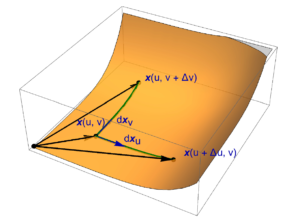A new version of Geometric Algebra for Electrical Engineers (V0.1.8) is now posted.  This fixes a number of issues in Chapter II on geometric calculus.  In particular, I had confused definitions of line, area, and volume integrals that were really the application of the fundamental theorem to such integrals.  This is now fixed, and the whole chapter is generally improved and clarified.

Notes so far for phy2403, Quantum Field Theory I

September 23, 2018 phy2403 No comments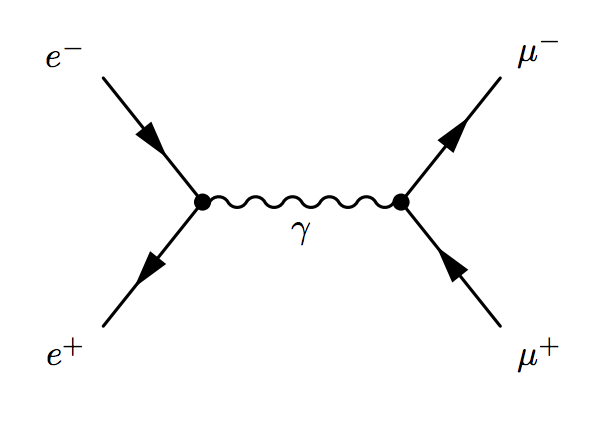Here’s an aggregate collection of notes for QFT I that I’ll update as the term progresses.  It contains very rough notes for the first 4 lectures (6 hours of material).

These notes also contain my (ungraded) problem set I solution from a few years ago.  I was originally going to take the class and had started preparing for it with some independent reading of the Professor’s class notes (Prof. Luke), and by doing problems he’d posted for a previous year.  I ended up changing my plans and took something else instead, probably to satisfy the graduation requirements for the M.Eng program.  It’s been so long since I’ve done those problems that I don’t even remember all the material that the problems covered (some of which we haven’t gotten to yet in class).

PHY2403H Quantum Field Theory. Lecture 4: Scalar action, least action principle, Euler-Lagrange equations for a field, canonical quantization. Taught by Prof. Erich Poppitz

DISCLAIMER: Very rough notes from class. May have some additional side notes, but otherwise probably barely edited.

These are notes for the UofT course PHY2403H, Quantum Field Theory I, taught by Prof. Erich Poppitz fall 2018.

Principles (cont.)

• Lorentz (Poincar\’e : Lorentz and spacetime translations)
• locality
• dimensional analysis
• gauge invariance

These are the requirements for an action. We postulated an action that had the form
\begin{equation}\label{eqn:qftLecture4:20}
\int d^d x \partial_\mu \phi \partial^\mu \phi,
\end{equation}
called the “Kinetic term”, which mimics $$\int dt \dot{q}^2$$ that we’d see in quantum or classical mechanics. In principle there exists an infinite number of local Poincar\’e invariant terms that we can write. Examples:

• $$\partial_\mu \phi \partial^\mu \phi$$
• $$\partial_\mu \phi \partial_\nu \partial^\nu \partial^\mu \phi$$
• $$\lr{\partial_\mu \phi \partial^\mu \phi}^2$$
• $$f(\phi) \partial_\mu \phi \partial^\mu \phi$$
• $$f(\phi, \partial_\mu \phi \partial^\mu \phi)$$
• $$V(\phi)$$

It turns out that nature (i.e. three spatial dimensions and one time dimension) is described by a finite number of terms. We will now utilize dimensional analysis to determine some of the allowed forms of the action for scalar field theories in $$d = 2, 3, 4, 5$$ dimensions. Even though the real world is only $$d = 4$$, some of the $$d < 4$$ theories are relevant in condensed matter studies, and $$d = 5$$ is just for fun (but also applies to string theories.)

With $$[x] \sim \inv{M}$$ in natural units, we must define $$[\phi]$$ such that the kinetic term is dimensionless in d spacetime dimensions

\begin{equation}\label{eqn:qftLecture4:40}
\begin{aligned}
[d^d x] &\sim \inv{M^d} \\
[\partial_\mu] &\sim M
\end{aligned}
\end{equation}

so it must be that
\begin{equation}\label{eqn:qftLecture4:60}
[\phi] = M^{(d-2)/2}
\end{equation}

It will be easier to characterize the dimensionality of any given term by the power of the mass units, that is

\begin{equation}\label{eqn:qftLecture4:80}
\begin{aligned}
[\text{mass}] &= 1 \\
[d^d x] &= -d \\
[\partial_\mu] &= 1 \\
[\phi] &= (d-2)/2 \\
[S] &= 0.
\end{aligned}
\end{equation}
Since the action is
\begin{equation}\label{eqn:qftLecture4:100}
S = \int d^d x \lr{ \LL(\phi, \partial_\mu \phi) },
\end{equation}
and because action had dimensions of $$\Hbar$$, so in natural units, it must be dimensionless, the Lagrangian density dimensions must be $$[d]$$. We will abuse language in QFT and call the Lagrangian density the Lagrangian.

$$d = 2$$

Because $$[\partial_\mu \phi \partial^\mu \phi ] = 2$$, the scalar field must be dimension zero, or in symbols
\begin{equation}\label{eqn:qftLecture4:120}
[\phi] = 0.
\end{equation}
This means that introducing any function $$f(\phi) = 1 + a \phi + b\phi^2 + c \phi^3 + \cdots$$ is also dimensionless, and
\begin{equation}\label{eqn:qftLecture4:140}
[f(\phi) \partial_\mu \phi \partial^\mu \phi ] = 2,
\end{equation}
for any $$f(\phi)$$. Another implication of this is that the a potential term in the Lagrangian $$[V(\phi)] = 0$$ needs a coupling constant of dimension 2. Letting $$\mu$$ have mass dimensions, our Lagrangian must have the form
\begin{equation}\label{eqn:qftLecture4:160}
f(\phi) \partial_\mu \phi \partial^\mu \phi + \mu^2 V(\phi).
\end{equation}
An infinite number of coupling constants of positive mass dimensions for $$V(\phi)$$ are also allowed. If we have higher order derivative terms, then we need to compensate for the negative mass dimensions. Example (still for $$d = 2$$).
\begin{equation}\label{eqn:qftLecture4:180}
\LL =
f(\phi) \partial_\mu \phi \partial^\mu \phi + \mu^2 V(\phi) + \inv{{\mu’}^2}\partial_\mu \phi \partial_\nu \partial^\nu \partial^\mu \phi + \lr{ \partial_\mu \phi \partial^\mu \phi }^2 \inv{\tilde{\mu}^2}.
\end{equation}
The last two terms, called \underline{couplings} (i.e. any non-kinetic term), are examples of terms with negative mass dimension. There is an infinite number of those in any theory in any dimension.

Definitions

• Couplings that are dimensionless are called (classically) marginal.
• Couplings that have positive mass dimension are called (classically) relevant.
• Couplings that have negative mass dimension are called (classically) irrelevant.

In QFT we are generally interested in the couplings that are measurable at long distances for some given energy. Classically irrelevant theories are generally not interesting in $$d > 2$$, so we are very lucky that we don’t live in three dimensional space. This means that we can get away with a finite number of classically marginal and relevant couplings in 3 or 4 dimensions. This was mentioned in the Wilczek’s article referenced in the class forum \footnote{There’s currently more in that article that I don’t understand than I do, so it is hard to find it terribly illuminating.}

Long distance physics in any dimension is described by the marginal and relevant couplings. The irrelevant couplings die off at low energy. In two dimensions, a priori, an infinite number of marginal and relevant couplings are possible. 2D is a bad place to live!

$$d = 3$$

Now we have
\begin{equation}\label{eqn:qftLecture4:200}
[\phi] = \inv{2}
\end{equation}
so that
\begin{equation}\label{eqn:qftLecture4:220}
[\partial_\mu \phi \partial^\mu \phi] = 3.
\end{equation}

A 3D Lagrangian could have local terms such as
\begin{equation}\label{eqn:qftLecture4:240}
\LL = \partial_\mu \phi \partial^\mu \phi + m^2 \phi^2 + \mu^{3/2} \phi^3 + \mu’ \phi^4
+ \lr{\mu”}{1/2} \phi^5
+ \lambda \phi^6.
\end{equation}
where $$m, \mu, \mu”$$ all have mass dimensions, and $$\lambda$$ is dimensionless. i.e. $$m, \mu, \mu”$$ are relevant, and $$\lambda$$ marginal. We stop at the sixth power, since any power after that will be irrelevant.

$$d = 4$$

Now we have
\begin{equation}\label{eqn:qftLecture4:260}
[\phi] = 1
\end{equation}
so that
\begin{equation}\label{eqn:qftLecture4:280}
[\partial_\mu \phi \partial^\mu \phi] = 4.
\end{equation}

In this number of dimensions $$\phi^k \partial_\mu \phi \partial^\mu$$ is an irrelevant coupling.

A 4D Lagrangian could have local terms such as
\begin{equation}\label{eqn:qftLecture4:300}
\LL = \partial_\mu \phi \partial^\mu \phi + m^2 \phi^2 + \mu \phi^3 + \lambda \phi^4.
\end{equation}
where $$m, \mu$$ have mass dimensions, and $$\lambda$$ is dimensionless. i.e. $$m, \mu$$ are relevant, and $$\lambda$$ is marginal.

$$d = 5$$

Now we have
\begin{equation}\label{eqn:qftLecture4:320}
[\phi] = \frac{3}{2},
\end{equation}
so that
\begin{equation}\label{eqn:qftLecture4:340}
[\partial_\mu \phi \partial^\mu \phi] = 5.
\end{equation}

A 5D Lagrangian could have local terms such as
\begin{equation}\label{eqn:qftLecture4:360}
\LL = \partial_\mu \phi \partial^\mu \phi + m^2 \phi^2 + \sqrt{\mu} \phi^3 + \inv{\mu’} \phi^4.
\end{equation}
where $$m, \mu, \mu’$$ all have mass dimensions. In 5D there are no marginal couplings. Dimension 4 is the last dimension where marginal couplings exist. In condensed matter physics 4D is called the “upper critical dimension”.

From the point of view of particle physics, all the terms in the Lagrangian must be the ones that are relevant at long distances.

Least action principle (classical field theory).

Now we want to study 4D scalar theories. We have some action
\begin{equation}\label{eqn:qftLecture4:380}
S[\phi] = \int d^4 x \LL(\phi, \partial_\mu \phi).
\end{equation}

Let’s keep an example such as the following in mind
\begin{equation}\label{eqn:qftLecture4:400}
\LL = \underbrace{\inv{2} \partial_\mu \phi \partial^\mu \phi}_{\text{Kinetic term}} – \underbrace{m^2 \phi – \lambda \phi^4}_{\text{all relevant and marginal couplings}}.
\end{equation}
The even powers can be justified by assuming there is some symmetry that kills the odd powered terms.

We will be integrating over a space time region such as that depicted in fig. 1, where a cylindrical spatial cross section is depicted that we allow to tend towards infinity. We demand that the field is fixed on the infinite spatial boundaries. The easiest way to demand that the field dies off on the spatial boundaries, that is
\begin{equation}\label{eqn:qftLecture4:420}
\lim_{\Abs{\Bx} \rightarrow \infty} \phi(\Bx) \rightarrow 0.
\end{equation}
The functional $$\phi(\Bx, t)$$ that obeys the boundary condition as stated extremizes $$S[\phi]$$.

Extremizing the action means that we seek $$\phi(\Bx, t)$$
\begin{equation}\label{eqn:qftLecture4:440}
\delta S[\phi] = 0 = S[\phi + \delta \phi] – S[\phi].
\end{equation}

How do we compute the variation?
\begin{equation}\label{eqn:qftLecture4:460}
\begin{aligned}
\delta S
&= \int d^d x \lr{ \LL(\phi + \delta \phi, \partial_\mu \phi + \partial_\mu \delta \phi) – \LL(\phi, \partial_\mu \phi) } \\
&= \int d^d x \lr{ \PD{\phi}{\LL} \delta \phi + \PD{(\partial_mu \phi)}{\LL} (\partial_\mu \delta \phi) } \\
&= \int d^d x \lr{ \PD{\phi}{\LL} \delta \phi
+ \partial_\mu \lr{ \PD{(\partial_mu \phi)}{\LL} \delta \phi}
– \lr{ \partial_\mu \PD{(\partial_mu \phi)}{\LL} } \delta \phi
} \\
&=
\int d^d x
\delta \phi
\lr{ \PD{\phi}{\LL}
– \partial_\mu \PD{(\partial_mu \phi)}{\LL} }
+ \int d^3 \sigma_\mu \lr{ \PD{(\partial_\mu \phi)}{\LL} \delta \phi }
\end{aligned}
\end{equation}

If we are explicit about the boundary term, we write it as
\begin{equation}\label{eqn:qftLecture4:480}
\int dt d^3 \Bx \partial_t \lr{ \PD{(\partial_t \phi)}{\LL} \delta \phi }
=
\int d^3 \Bx \evalrange{ \PD{(\partial_t \phi)}{\LL} \delta \phi }{t = -T}{t = T}
– \int dt d^2 \BS \cdot \lr{ \PD{(\spacegrad \phi)}{\LL} \delta \phi }.
\end{equation}
but $$\delta \phi = 0$$ at $$t = \pm T$$ and also at the spatial boundaries of the integration region.

This leaves
\begin{equation}\label{eqn:qftLecture4:500}
\delta S[\phi] = \int d^d x \delta \phi
\lr{ \PD{\phi}{\LL} – \partial_\mu \PD{(\partial_mu \phi)}{\LL} } = 0 \forall \delta \phi.
\end{equation}
That is

\begin{equation}\label{eqn:qftLecture4:540}
\boxed{
\PD{\phi}{\LL} – \partial_\mu \PD{(\partial_mu \phi)}{\LL} = 0.
}
\end{equation}

This are the Euler-Lagrange equations for a single scalar field.

Returning to our sample scalar Lagrangian
\begin{equation}\label{eqn:qftLecture4:560}
\LL = \inv{2} \partial_\mu \phi \partial^\mu \phi – \inv{2} m^2 \phi^2 – \frac{\lambda}{4} \phi^4.
\end{equation}
This example is related to the Ising model which has a $$\phi \rightarrow -\phi$$ symmetry. Applying the Euler-Lagrange equations, we have
\begin{equation}\label{eqn:qftLecture4:580}
\PD{\phi}{\LL} = -m^2 \phi – \lambda \phi^3,
\end{equation}
and
\begin{equation}\label{eqn:qftLecture4:600}
\begin{aligned}
\PD{(\partial_\mu \phi)}{\LL}
&=
\PD{(\partial_\mu \phi)}{} \lr{
\inv{2} \partial_\nu \phi \partial^\nu \phi } \\
&=
\inv{2} \partial^\nu \phi
\PD{(\partial_\mu \phi)}{}
\partial_\nu \phi
+
\inv{2} \partial_\nu \phi
\PD{(\partial_\mu \phi)}{}
\partial_\alpha \phi g^{\nu\alpha} \\
&=
\inv{2} \partial^\mu \phi
+
\inv{2} \partial_\nu \phi g^{\nu\mu} \\
&=
\partial^\mu \phi
\end{aligned}
\end{equation}
so we have
\begin{equation}\label{eqn:qftLecture4:620}
\begin{aligned}
0
&=
\PD{\phi}{\LL} -\partial_\mu
\PD{(\partial_\mu \phi)}{\LL} \\
&=
-m^2 \phi – \lambda \phi^3 – \partial_\mu \partial^\mu \phi.
\end{aligned}
\end{equation}

For $$\lambda = 0$$, the free field theory limit, this is just
\begin{equation}\label{eqn:qftLecture4:640}
\partial_\mu \partial^\mu \phi + m^2 \phi = 0.
\end{equation}
Written out from the observer frame, this is
\begin{equation}\label{eqn:qftLecture4:660}
(\partial_t)^2 \phi – \spacegrad^2 \phi + m^2 \phi = 0.
\end{equation}

With a non-zero mass term
\begin{equation}\label{eqn:qftLecture4:680}
\lr{ \partial_t^2 – \spacegrad^2 + m^2 } \phi = 0,
\end{equation}
is called the Klein-Gordan equation.

If we also had $$m = 0$$ we’d have
\begin{equation}\label{eqn:qftLecture4:700}
\lr{ \partial_t^2 – \spacegrad^2 } \phi = 0,
\end{equation}
which is the wave equation (for a massless free field). This is also called the D’Alembert equation, which is familiar from electromagnetism where we have
\begin{equation}\label{eqn:qftLecture4:720}
\begin{aligned}
\lr{ \partial_t^2 – \spacegrad^2 } \BE &= 0 \\
\lr{ \partial_t^2 – \spacegrad^2 } \BB &= 0,
\end{aligned}
\end{equation}
in a source free region.

Canonical quantization.

\begin{equation}\label{eqn:qftLecture4:740}
\LL = \inv{2} \dot{q} – \frac{\omega^2}{2} q^2
\end{equation}
This has solution $$\ddot{q} = – \omega^2 q$$.

Let
\begin{equation}\label{eqn:qftLecture4:760}
p = \PD{\dot{q}}{\LL} = \dot{q}
\end{equation}
\begin{equation}\label{eqn:qftLecture4:780}
H(p,q) = \evalbar{p \dot{q} – \LL}{\dot{q}(p, q)}
= p p – \inv{2} p^2 + \frac{\omega^2}{2} q^2 = \frac{p^2}{2} + \frac{\omega^2}{2} q^2
\end{equation}

In QM we quantize by mapping Poisson brackets to commutators.
\begin{equation}\label{eqn:qftLecture4:800}
\antisymmetric{\hatp}{\hat{q}} = -i
\end{equation}
One way to represent is to say that states are $$\Psi(\hat{q})$$, a wave function, $$\hat{q}$$ acts by $$q$$
\begin{equation}\label{eqn:qftLecture4:820}
\hat{q} \Psi = q \Psi(q)
\end{equation}
With
\begin{equation}\label{eqn:qftLecture4:840}
\hatp = -i \PD{q}{},
\end{equation}
so
\begin{equation}\label{eqn:qftLecture4:860}
\antisymmetric{ -i \PD{q}{} } { q} = -i
\end{equation}

Let’s introduce an explicit space time split. We’ll write
\begin{equation}\label{eqn:qftLecture4:880}
L = \int d^3 x \lr{
\inv{2} (\partial_0 \phi(\Bx, t))^2 – \inv{2} \lr{ \spacegrad \phi(\Bx, t) }^2 – \frac{m^2}{2} \phi
},
\end{equation}
so that the action is
\begin{equation}\label{eqn:qftLecture4:900}
S = \int dt L.
\end{equation}
The dynamical variables are $$\phi(\Bx)$$. We define
\begin{equation}\label{eqn:qftLecture4:920}
\begin{aligned}
\pi(\Bx, t) = \frac{\delta L}{\delta (\partial_0 \phi(\Bx, t))}
&=
\partial_0 \phi(\Bx, t) \\
&=
\dot{\phi}(\Bx, t),
\end{aligned}
\end{equation}
called the canonical momentum, or the momentum conjugate to $$\phi(\Bx, t)$$. Why $$\delta$$? Has to do with an implicit Dirac function to eliminate the integral?

\begin{equation}\label{eqn:qftLecture4:940}
\begin{aligned}
H
&= \int d^3 x \evalbar{\lr{ \pi(\bar{\Bx}, t) \dot{\phi}(\bar{\Bx}, t) – L }}{\dot{\phi}(\bar{\Bx}, t) = \pi(x, t) } \\
&= \int d^3 x \lr{ (\pi(\Bx, t))^2 – \inv{2} (\pi(\Bx, t))^2 + \inv{2} (\spacegrad \phi)^2 + \frac{m}{2} \phi^2 },
\end{aligned}
\end{equation}
or
\begin{equation}\label{eqn:qftLecture4:960}
H
= \int d^3 x \lr{ \inv{2} (\pi(\Bx, t))^2 + \inv{2} (\spacegrad \phi(\Bx, t))^2 + \frac{m}{2} (\phi(\Bx, t))^2 }
\end{equation}

In analogy to the momentum, position commutator in QM
\begin{equation}\label{eqn:qftLecture4:1000}
\antisymmetric{\hat{p}_i}{\hat{q}_j} = -i \delta_{ij},
\end{equation}
we “quantize” the scalar field theory by promoting $$\pi, \phi$$ to operators and insisting that they also obey a commutator relationship
\begin{equation}\label{eqn:qftLecture4:980}
\antisymmetric{\pi(\Bx, t)}{\phi(\By, t)} = -i \delta^3(\Bx – \By).
\end{equation}

References

 Frank Wilczek. Fundamental constants. arXiv preprint arXiv:0708.4361, 2007. URL https://arxiv.org/abs/0708.4361.

PHY2403H Quantum Field Theory. Lecture 3: Lorentz transformations and a scalar action. Taught by Prof. Erich Poppitz

September 18, 2018 phy2403 No comments , ,

DISCLAIMER: Very rough notes from class. Some additional side notes, but otherwise barely edited.

These are notes for the UofT course PHY2403H, Quantum Field Theory, taught by Prof. Erich Poppitz.

Determinant of Lorentz transformations

We require that Lorentz transformations leave the dot product invariant, that is $$x \cdot y = x’ \cdot y’$$, or
\begin{equation}\label{eqn:qftLecture3:20}
x^\mu g_{\mu\nu} y^\nu = {x’}^\mu g_{\mu\nu} {y’}^\nu.
\end{equation}
Explicitly, with coordinate transformations
\begin{equation}\label{eqn:qftLecture3:40}
\begin{aligned}
{x’}^\mu &= {\Lambda^\mu}_\rho x^\rho \\
{y’}^\mu &= {\Lambda^\mu}_\rho y^\rho
\end{aligned}
\end{equation}
such a requirement is equivalent to demanding that
\begin{equation}\label{eqn:qftLecture3:500}
\begin{aligned}
x^\mu g_{\mu\nu} y^\nu
&=
{\Lambda^\mu}_\rho x^\rho
g_{\mu\nu}
{\Lambda^\nu}_\kappa y^\kappa \\
&=
x^\mu
{\Lambda^\alpha}_\mu
g_{\alpha\beta}
{\Lambda^\beta}_\nu
y^\nu,
\end{aligned}
\end{equation}
or
\begin{equation}\label{eqn:qftLecture3:60}
g_{\mu\nu}
=
{\Lambda^\alpha}_\mu
g_{\alpha\beta}
{\Lambda^\beta}_\nu
\end{equation}

multiplying by the inverse we find
\begin{equation}\label{eqn:qftLecture3:200}
\begin{aligned}
g_{\mu\nu}
{\lr{\Lambda^{-1}}^\nu}_\lambda
&=
{\Lambda^\alpha}_\mu
g_{\alpha\beta}
{\Lambda^\beta}_\nu
{\lr{\Lambda^{-1}}^\nu}_\lambda \\
&=
{\Lambda^\alpha}_\mu
g_{\alpha\lambda} \\
&=
g_{\lambda\alpha}
{\Lambda^\alpha}_\mu.
\end{aligned}
\end{equation}
This is now amenable to expressing in matrix form
\begin{equation}\label{eqn:qftLecture3:220}
\begin{aligned}
(G \Lambda^{-1})_{\mu\lambda}
&=
(G \Lambda)_{\lambda\mu} \\
&=
((G \Lambda)^\T)_{\mu\lambda} \\
&=
(\Lambda^\T G)_{\mu\lambda},
\end{aligned}
\end{equation}
or
\begin{equation}\label{eqn:qftLecture3:80}
G \Lambda^{-1}
=
(G \Lambda)^\T.
\end{equation}

Taking determinants (using the normal identities for products of determinants, determinants of transposes and inverses), we find
\begin{equation}\label{eqn:qftLecture3:100}
det(G)
det(\Lambda^{-1})
=
det(G) det(\Lambda),
\end{equation}
or
\begin{equation}\label{eqn:qftLecture3:120}
det(\Lambda)^2 = 1,
\end{equation}
or
$$det(\Lambda)^2 = \pm 1$$. We will generally ignore the case of reflections in spacetime that have a negative determinant.

Smart-alec Peeter pointed out after class last time that we can do the same thing easier in matrix notation
\begin{equation}\label{eqn:qftLecture3:140}
\begin{aligned}
x’ &= \Lambda x \\
y’ &= \Lambda y
\end{aligned}
\end{equation}
where
\begin{equation}\label{eqn:qftLecture3:160}
\begin{aligned}
x’ \cdot y’
&=
(x’)^\T G y’ \\
&=
x^\T \Lambda^\T G \Lambda y,
\end{aligned}
\end{equation}
which we require to be $$x \cdot y = x^\T G y$$ for all four vectors $$x, y$$, that is
\begin{equation}\label{eqn:qftLecture3:180}
\Lambda^\T G \Lambda = G.
\end{equation}
We can find the result \ref{eqn:qftLecture3:120} immediately without having to first translate from index notation to matrices.

Field theory

The electrostatic potential is an example of a scalar field $$\phi(\Bx)$$ unchanged by SO(3) rotations
\begin{equation}\label{eqn:qftLecture3:240}
\Bx \rightarrow \Bx’ = O \Bx,
\end{equation}
that is
\begin{equation}\label{eqn:qftLecture3:260}
\phi'(\Bx’) = \phi(\Bx).
\end{equation}
Here $$\phi'(\Bx’)$$ is the value of the (electrostatic) scalar potential in a primed frame.

However, the electrostatic field is not invariant under Lorentz transformation.
We postulate that there is some scalar field
\begin{equation}\label{eqn:qftLecture3:280}
\phi'(x’) = \phi(x),
\end{equation}
where $$x’ = \Lambda x$$ is an SO(1,3) transformation. There are actually no stable particles (fields that persist at long distances) described by Lorentz scalar fields, although there are some unstable scalar fields such as the Higgs, Pions, and Kaons. However, much of our homework and discussion will be focused on scalar fields, since they are the easiest to start with.

We need to first understand how derivatives $$\partial_\mu \phi(x)$$ transform. Using the chain rule
\begin{equation}\label{eqn:qftLecture3:300}
\begin{aligned}
\PD{x^\mu}{\phi(x)}
&=
\PD{x^\mu}{\phi'(x’)} \\
&=
\PD{{x’}^\nu}{\phi'(x’)}
\PD{{x}^\mu}{{x’}^\nu} \\
&=
\PD{{x’}^\nu}{\phi'(x’)}
\partial_\mu \lr{
{\Lambda^\nu}_\rho x^\rho
} \\
&=
\PD{{x’}^\nu}{\phi'(x’)}
{\Lambda^\nu}_\mu \\
&=
\PD{{x’}^\nu}{\phi(x)}
{\Lambda^\nu}_\mu.
\end{aligned}
\end{equation}
Multiplying by the inverse $${\lr{\Lambda^{-1}}^\mu}_\kappa$$ we get
\begin{equation}\label{eqn:qftLecture3:320}
\PD{{x’}^\kappa}{}
=
{\lr{\Lambda^{-1}}^\mu}_\kappa \PD{x^\mu}{}
\end{equation}

This should be familiar to you, and is an analogue of the transformation of the
\begin{equation}\label{eqn:qftLecture3:340}
=
\end{equation}

Actions

We will start with a classical action, and quantize to determine a QFT. In mechanics we have the particle position $$q(t)$$, which is a classical field in 1+0 time and space dimensions. Our action is
\begin{equation}\label{eqn:qftLecture3:360}
S
= \int dt \LL(t)
= \int dt \lr{
\inv{2} \dot{q}^2 – V(q)
}.
\end{equation}
This action depends on the position of the particle that is local in time. You could imagine that we have a more complex action where the action depends on future or past times
\begin{equation}\label{eqn:qftLecture3:380}
S
= \int dt’ q(t’) K( t’ – t ),
\end{equation}
but we don’t seem to find such actions in classical mechanics.

Principles determining the form of the action.

• relativity (action is invariant under Lorentz transformation)
• locality (action depends on fields and the derivatives at given $$(t, \Bx)$$.
• Gauge principle (the action should be invariant under gauge transformation). We won’t discuss this in detail right now since we will start with studying scalar fields.
Recall that for Maxwell’s equations a gauge transformation has the form
\begin{equation}\label{eqn:qftLecture3:520}
\phi \rightarrow \phi + \dot{\chi}, \BA \rightarrow \BA – \spacegrad \chi.
\end{equation}

Suppose we have a real scalar field $$\phi(x)$$ where $$x \in \mathbb{R}^{1,d-1}$$. We will be integrating over space and time $$\int dt d^{d-1} x$$ which we will write as $$\int d^d x$$. Our action is
\begin{equation}\label{eqn:qftLecture3:400}
S = \int d^d x \lr{ \text{Some action density to be determined } }
\end{equation}
The analogue of $$\dot{q}^2$$ is
\begin{equation}\label{eqn:qftLecture3:420}
\begin{aligned}
\lr{ \PD{x^\mu}{\phi} }
\lr{ \PD{x^\nu}{\phi} }
g^{\mu\nu}
&=
(\partial_\mu \phi) (\partial_\nu \phi) g^{\mu\nu} \\
&= \partial^\mu \phi \partial_\mu \phi.
\end{aligned}
\end{equation}
This has both time and spatial components, that is
\begin{equation}\label{eqn:qftLecture3:440}
\partial^\mu \phi \partial_\mu \phi =
\end{equation}
so the desired simplest scalar action is
\begin{equation}\label{eqn:qftLecture3:460}
S = \int d^d x \lr{ \dotphi^2 – (\spacegrad \phi)^2 }.
\end{equation}
The measure transforms using a Jacobian, which we have seen is the Lorentz transform matrix, and has unit determinant
\begin{equation}\label{eqn:qftLecture3:480}
d^d x’ = d^d x \Abs{ det( \Lambda^{-1} ) } = d^d x.
\end{equation}

Question: Four vector form of the Maxwell gauge transformation.

Show that the transformation
\begin{equation}\label{eqn:qftLecture3:580}
A^\mu \rightarrow A^\mu + \partial^\mu \chi
\end{equation}
is the desired four-vector form of the gauge transformation \ref{eqn:qftLecture3:520}, that is
\begin{equation}\label{eqn:qftLecture3:540}
\begin{aligned}
j^\nu
&= \partial_\mu {F’}^{\mu\nu} \\
&= \partial_\mu F^{\mu\nu}.
\end{aligned}
\end{equation}
Also relate this four-vector gauge transformation to the spacetime split.

\begin{equation}\label{eqn:qftLecture3:560}
\begin{aligned}
\partial_\mu {F’}^{\mu\nu}
&=
\partial_\mu \lr{ \partial^\mu {A’}^\nu – \partial_\nu {A’}^\mu } \\
&=
\partial_\mu \lr{
\partial^\mu \lr{ A^\nu + \partial^\nu \chi }
– \partial_\nu \lr{ A^\mu + \partial^\mu \chi }
} \\
&=
\partial_\mu {F}^{\mu\nu}
+
\partial_\mu \partial^\mu \partial^\nu \chi

\partial_\mu \partial^\nu \partial^\mu \chi \\
&=
\partial_\mu {F}^{\mu\nu},
\end{aligned}
\end{equation}
by equality of mixed partials. Expanding \ref{eqn:qftLecture3:580} explicitly we find
\begin{equation}\label{eqn:qftLecture3:600}
{A’}^\mu = A^\mu + \partial^\mu \chi,
\end{equation}
which is
\begin{equation}\label{eqn:qftLecture3:620}
\begin{aligned}
\phi’ = {A’}^0 &= A^0 + \partial^0 \chi = \phi + \dot{\chi} \\
\BA’ \cdot \Be_k = {A’}^k &= A^k + \partial^k \chi = \lr{ \BA – \spacegrad \chi } \cdot \Be_k.
\end{aligned}
\end{equation}
The last of which can be written in vector notation as $$\BA’ = \BA – \spacegrad \chi$$.

UofT QFT Fall 2018 Lecture 2. Units, scales, and Lorentz transformations. Taught by Prof. Erich Poppitz

September 17, 2018 phy2403 No comments , , ,

Natural units.

\begin{equation}\label{eqn:qftLecture2:20}
\begin{aligned}
[\Hbar] &= [\text{action}] = M \frac{L^2}{T^2} T = \frac{M L^2}{T} \\
&= [\text{velocity}] = \frac{L}{T} \\
& [\text{energy}] = M \frac{L^2}{T^2}.
\end{aligned}
\end{equation}

Setting $$c = 1$$ means
\begin{equation}\label{eqn:qftLecture2:240}
\frac{L}{T} = 1
\end{equation}
and setting $$\Hbar = 1$$ means
\begin{equation}\label{eqn:qftLecture2:260}
[\Hbar] = [\text{action}] = M L {\frac{L}{T}} = M L
\end{equation}
therefore
\begin{equation}\label{eqn:qftLecture2:280}
[L] = \inv{\text{mass}}
\end{equation}
and
\begin{equation}\label{eqn:qftLecture2:300}
[\text{energy}] = M {\frac{L^2}{T^2}} = \text{mass}\, \text{eV}
\end{equation}

Summary

• $$\text{energy} \sim \text{eV}$$
• $$\text{distance} \sim \inv{M}$$
• $$\text{time} \sim \inv{M}$$

From:
\begin{equation}\label{eqn:qftLecture2:320}
\alpha = \frac{e^2}{4 \pi {\Hbar c}}
\end{equation}
which is dimensionless ($$1/137$$), so electric charge is dimensionless.

Some useful numbers in natural units

\begin{equation}\label{eqn:qftLecture2:40}
\begin{aligned}
m_\txte &\sim 10^{-27} \text{g} \sim 0.5 \text{MeV} \\
m_\txtp &\sim 2000 m_\txte \sim 1 \text{GeV} \\
m_\pi &\sim 140 \text{MeV} \\
m_\mu &\sim 105 \text{MeV} \\
\Hbar c &\sim 200 \text{MeV} \,\text{fm} = 1
\end{aligned}
\end{equation}

Gravity

Interaction energy of two particles

\begin{equation}\label{eqn:qftLecture2:60}
G_\txtN \frac{m_1 m_2}{r}
\end{equation}

\begin{equation}\label{eqn:qftLecture2:80}
[\text{energy}] \sim [G_\txtN] \frac{M^2}{L}
\end{equation}

\begin{equation}\label{eqn:qftLecture2:100}
[G_\txtN]
\sim
[\text{energy}] \frac{L}{M^2}
\end{equation}

but energy x distance is dimensionless (action) in our units

\begin{equation}\label{eqn:qftLecture2:120}
[G_\txtN]
\sim
{\text{dimensionless}}{M^2}
\end{equation}

\begin{equation}\label{eqn:qftLecture2:140}
\frac{G_\txtN}{\Hbar c} \sim \inv{M^2} \sim \frac{1}{10^{20} \text{GeV}}
\end{equation}

Planck mass

\begin{equation}\label{eqn:qftLecture2:160}
M_{\text{Planck}} \sim \sqrt{\frac{\Hbar c}{G_\txtN}}
\sim 10^{-4} g \sim \inv{\lr{10^{20} \text{GeV}}^2}
\end{equation}

We can revisit the scale diagram from last lecture in terms of MeV mass/energy values, as sketched in fig. 1.

At the classical electron radius scale, we consider phenomena such as back reaction of radiation, the self energy of electrons. At the Compton wavelength we have to allow for production of multiple particle pairs. At Bohr radius scales we must start using QM instead of classical mechanics.

Cross section.

Verbal discussion of cross section, not captured in these notes. Roughly, the cross section sounds like the number of events per unit time, related to the flux of some source through an area.

We’ll compute the cross section of a number of different systems in this course. The cross section is relevant in scattering such as the electron-electron scattering sketched in fig. 2.

We assume that QED is highly relativistic. In natural units, our scale factor is basically the square of the electric charge
\begin{equation}\label{eqn:qftLecture2:180}
\alpha \sim e^2,
\end{equation}
so the cross section has the form
\begin{equation}\label{eqn:qftLecture2:200}
\sigma \sim \frac{\alpha^2}{E^2} \lr{ 1 + O(\alpha) + O(\alpha^2) + \cdots }
\end{equation}

In gravity we could consider scattering of electrons, where $$G_\txtN$$ takes the place of $$\alpha$$. However, $$G_\txtN$$ has dimensions.

For electron-electron scattering due to gravitons

\begin{equation}\label{eqn:qftLecture2:220}
\sigma \sim \frac{G_\txtN^2 E^2}{1 + G_\txtN E^2 + \cdots }
\end{equation}

Now the cross section grows with energy. This will cause some problems (violating unitarity: probabilities greater than 1!) when $$O(G_\txtN E^2) = 1$$.

In any quantum field theories when the coupling constant is not-dimensionless we have the same sort of problems at some scale.

The point is that we can get far considering just dimensional analysis.

If the coupling constant has a dimension $$(1/\text{mass})^N\,, N > 0$$, then unitarity will be violated at high energy. One such theory is the Fermi theory of beta decay (electro-weak theory), which had a coupling constant with dimensions inverse-mass-squared. The relevant scale for beta decay was 4 Fermi, or $$G_\txtF \sim (1/{100 \text{GeV}})^2$$. This was the motivation for introducing the Higgs theory, which was motivated by restoring unitarity.

Lorentz transformations.

The goal, perhaps not for today, is to study the simplest (relativistic) scalar field theory. First studied classically, and then consider such a quantum field theory.

How is relativity implemented when we write the Lagrangian and action?

Our first step must be to consider Lorentz transformations and the Lorentz group.

Spacetime (Minkowski space) is \R{3,1} (or \R{d-1,1}). Our coordinates are

\begin{equation}\label{eqn:qftLecture2:340}
(c t, x^1, x^2, x^3) = (c t, \Br).
\end{equation}

Here, we’ve scaled the time scale by $$c$$ so that we measure time and space in the same dimensions. We write this as

\begin{equation}\label{eqn:qftLecture2:360}
x^\mu = (x^0, x^1, x^2, x^3),
\end{equation}

where $$\mu = 0, 1, 2, 3$$, and call this a “4-vector”. These are called the space-time coordinates of an event, which tell us where and when an event occurs.

For two events whose spacetime coordinates differ by $$dx^0, dx^1, dx^2, dx^3$$ we introduce the notion of a space time \underline{interval}

\begin{equation}\label{eqn:qftLecture2:380}
\begin{aligned}
ds^2
&= c^2 dt^2
– (dx^1)^2
– (dx^2)^2
– (dx^3)^2 \\
&=
\sum_{\mu, \nu = 0}^3 g_{\mu\nu} dx^\mu dx^\nu
\end{aligned}
\end{equation}

Here $$g_{\mu\nu}$$ is the Minkowski space metric, an object with two indexes that run from 0-3. i.e. this is a diagonal matrix

\begin{equation}\label{eqn:qftLecture2:400}
g_{\mu\nu} \sim
\begin{bmatrix}
1 & 0 & 0 & 0 \\
0 & -1 & 0 & 0 \\
0 & 0 & -1 & 0 \\
0 & 0 & 0 & -1 \\
\end{bmatrix}
\end{equation}

i.e.
\begin{equation}\label{eqn:qftLecture2:420}
\begin{aligned}
g_{00} &= 1 \\
g_{11} &= -1 \\
g_{22} &= -1 \\
g_{33} &= -1 \\
\end{aligned}
\end{equation}

We will use the Einstein summation convention, where any repeated upper and lower indexes are considered summed over. That is \ref{eqn:qftLecture2:380} is written with an implied sum
\begin{equation}\label{eqn:qftLecture2:440}
ds^2 = g_{\mu\nu} dx^\mu dx^\nu.
\end{equation}

Explicit expansion:
\begin{equation}\label{eqn:qftLecture2:460}
\begin{aligned}
ds^2
&= g_{\mu\nu} dx^\mu dx^\nu \\
&=
g_{00} dx^0 dx^0
+g_{11} dx^1 dx^1
+g_{22} dx^2 dx^2
+g_{33} dx^3 dx^3
&=
(1) dx^0 dx^0
+ (-1) dx^1 dx^1
+ (-1) dx^2 dx^2
+ (-1) dx^3 dx^3.
\end{aligned}
\end{equation}

Recall that rotations (with orthogonal matrix representations) are transformations that leave the dot product unchanged, that is

\begin{equation}\label{eqn:qftLecture2:480}
\begin{aligned}
(R \Bx) \cdot (R \By)
&= \Bx^\T R^\T R \By \\
&= \Bx^\T \By \\
&= \Bx \cdot \By,
\end{aligned}
\end{equation}

where $$R$$ is a rotation orthogonal 3×3 matrix. The set of such transformations that leave the dot product unchanged have orthonormal matrix representations $$R^\T R = 1$$. We call the set of such transformations that have unit determinant the SO(3) group.

We call a Lorentz transformation, if it is a linear transformation acting on 4 vectors that leaves the spacetime interval (i.e. the inner product of 4 vectors) invariant. That is, a transformation that leaves
\begin{equation}\label{eqn:qftLecture2:500}
x^\mu y^\nu g_{\mu\nu} = x^0 y^0 – x^1 y^1 – x^2 y^2 – x^3 y^3
\end{equation}
unchanged.

Suppose that transformation has a 4×4 matrix form

\begin{equation}\label{eqn:qftLecture2:520}
{x’}^\mu = {\Lambda^\mu}_\nu x^\nu
\end{equation}

For an example of a possible $$\Lambda$$, consider the transformation sketched in fig. 3.

We know that boost has the form
\begin{equation}\label{eqn:qftLecture2:540}
\begin{aligned}
x &= \frac{x’ + v t’}{\sqrt{1 – v^2/c^2}} \\
y &= y’ \\
z &= z’ \\
t &= \frac{t’ + (v/c^2) x’}{\sqrt{1 – v^2/c^2}} \\
\end{aligned}
\end{equation}
(this is a boost along the x-axis, not y as I’d drawn),
or
\begin{equation}\label{eqn:qftLecture2:560}
\begin{bmatrix}
c t \\
x \\
y \\
z
\end{bmatrix}
=
\begin{bmatrix}
\inv{\sqrt{1 – v^2/c^2}} & \frac{v/c}{\sqrt{1 – v^2/c^2}} & 0 & 0 \\
\frac{v/c}{\sqrt{1 – v^2/c^2}} & \frac{1}{\sqrt{1 – v^2/c^2}} & 0 & 0 \\
0 & 0 & 1 & 0 \\
0 & 0 & 0 & 1 \\
\end{bmatrix}
\begin{bmatrix}
c t’ \\
x’ \\
y’ \\
z’
\end{bmatrix}
\end{equation}

Other examples include rotations ($${\lambda^0}_0 = 1$$ zeros in $${\lambda^0}_k, {\lambda^k}_0$$, and a rotation matrix in the remainder.)

Back to Lorentz transformations ($$\text{SO}(1,3)^+$$), let
\begin{equation}\label{eqn:qftLecture2:600}
\begin{aligned}
{x’}^\mu &= {\Lambda^\mu}_\nu x^\nu \\
{y’}^\kappa &= {\Lambda^\kappa}_\rho y^\rho
\end{aligned}
\end{equation}

The dot product
\begin{equation}\label{eqn:qftLecture2:620}
g_{\mu \kappa}
{x’}^\mu
{y’}^\kappa
=
g_{\mu \kappa}
{\Lambda^\mu}_\nu
{\Lambda^\kappa}_\rho
x^\nu
y^\rho
=
g_{\nu\rho}
x^\nu
y^\rho,
\end{equation}
where the last step introduces the invariance requirement of the transformation. That is

\begin{equation}\label{eqn:qftLecture2:640}
\boxed{
g_{\nu\rho}
=
g_{\mu \kappa}
{\Lambda^\mu}_\nu
{\Lambda^\kappa}_\rho.
}
\end{equation}

Upper and lower indexes

We’ve defined

\begin{equation}\label{eqn:qftLecture2:660}
x^\mu = (t, x^1, x^2, x^3)
\end{equation}

We could also define a four vector with lower indexes
\begin{equation}\label{eqn:qftLecture2:680}
x_\nu = g_{\nu\mu} x^\mu = (t, -x^1, -x^2, -x^3).
\end{equation}
That is
\begin{equation}\label{eqn:qftLecture2:700}
\begin{aligned}
x_0 &= x^0 \\
x_1 &= -x^1 \\
x_2 &= -x^2 \\
x_3 &= -x^3.
\end{aligned}
\end{equation}

which allows us to write the dot product as simply $$x^\mu y_\mu$$.

We can also define a metric tensor with upper indexes

\begin{equation}\label{eqn:qftLecture2:401}
g^{\mu\nu} \sim
\begin{bmatrix}
1 & 0 & 0 & 0 \\
0 & -1 & 0 & 0 \\
0 & 0 & -1 & 0 \\
0 & 0 & 0 & -1 \\
\end{bmatrix}
\end{equation}
This is the inverse matrix of $$g_{\mu\nu}$$, and it satisfies
\begin{equation}\label{eqn:qftLecture2:720}
g^{\mu \nu} g_{\nu\rho} = {\delta^\mu}_\rho
\end{equation}

Exercise: Check:
\begin{equation}\label{eqn:qftLecture2:740}
\begin{aligned}
g_{\mu\nu} x^\mu y^\nu
&= x_\nu y^\nu \\
&= x^\nu y_\nu \\
&= g^{\mu\nu} x_\mu y_\nu \\
&= {\delta^\mu}_\nu x_\mu y^\nu.
\end{aligned}
\end{equation}

Class ended around this point, but it appeared that we were heading this direction:

Returning to the Lorentz invariant and multiplying both sides of
\ref{eqn:qftLecture2:640} with an inverse Lorentz transformation $$\Lambda^{-1}$$, we find
\begin{equation}\label{eqn:qftLecture2:760}
\begin{aligned}
g_{\nu\rho}
{\lr{\Lambda^{-1}}^\rho}_\alpha
&=
g_{\mu \kappa}
{\Lambda^\mu}_\nu
{\Lambda^\kappa}_\rho
{\lr{\Lambda^{-1}}^\rho}_\alpha \\
&=
g_{\mu \kappa}
{\Lambda^\mu}_\nu
{\delta^\kappa}_\alpha \\
&=
g_{\mu \alpha}
{\Lambda^\mu}_\nu,
\end{aligned}
\end{equation}
or
\begin{equation}\label{eqn:qftLecture2:780}
\lr{\Lambda^{-1}}_{\nu \alpha} = \Lambda_{\alpha \nu}.
\end{equation}
This is clearly analogous to $$R^\T = R^{-1}$$, although the index notation obscures things considerably. Prof. Poppitz said that next week this would all lead to showing that the determinant of any Lorentz transformation was $$\pm 1$$.

For what it’s worth, it seems to me that this index notation makes life a lot harder than it needs to be, at least for a matrix related question (i.e. determinant of the transformation). In matrix/column-(4)-vector notation, let $$x’ = \Lambda x, y’ = \Lambda y$$ be two four vector transformations, then
\begin{equation}\label{eqn:qftLecture2:800}
x’ \cdot y’ = {x’}^T G y’ = (\Lambda x)^T G \Lambda y = x^T ( \Lambda^T G \Lambda) y = x^T G y.
\end{equation}
so
\begin{equation}\label{eqn:qftLecture2:820}
\boxed{
\Lambda^T G \Lambda = G.
}
\end{equation}
Taking determinants of both sides gives $$-(det(\Lambda))^2 = -1$$, and thus $$det(\Lambda) = \pm 1$$.

Awesome bookshelves in my home office space

September 15, 2018 Incoherent ramblings No comments , ,

Sofia and I spend a large part of the day installing a set of four Ikea Liatorp bookshelves in my office today. The shelves fit pretty much perfectly, with a 1/4″ gap on each side. In fact, to get them to fit we had to take the baseboards and window casings off, but I’ll put in new ones butting up nicely to the shelves. When we eventually sell the house, the buyer better be interested in bookshelves, because these are a permanent feature of the house now!

The Liatorp model shelves are nicely engineered.  There are easy access leveling pegs, they join together nicely, and the backer board uses screws with pre-drilled holes in exactly the right places, plug some other plugs that hold the backer in place (far superior to the Billy model!)

Here’s a view of the whole shelf unit, which is loaded bottom heavy since the top shelves are spaces closer at the moment:I had a lot of fun moving books down from the bedroom bookshelves, and have moved most of the non-fiction content.  I was really pleased that I can mostly group my books in logical categories:

• Statistics and probability, with a couple German books and a dictionary too big to fit with the language material.
• Calculus and engineering: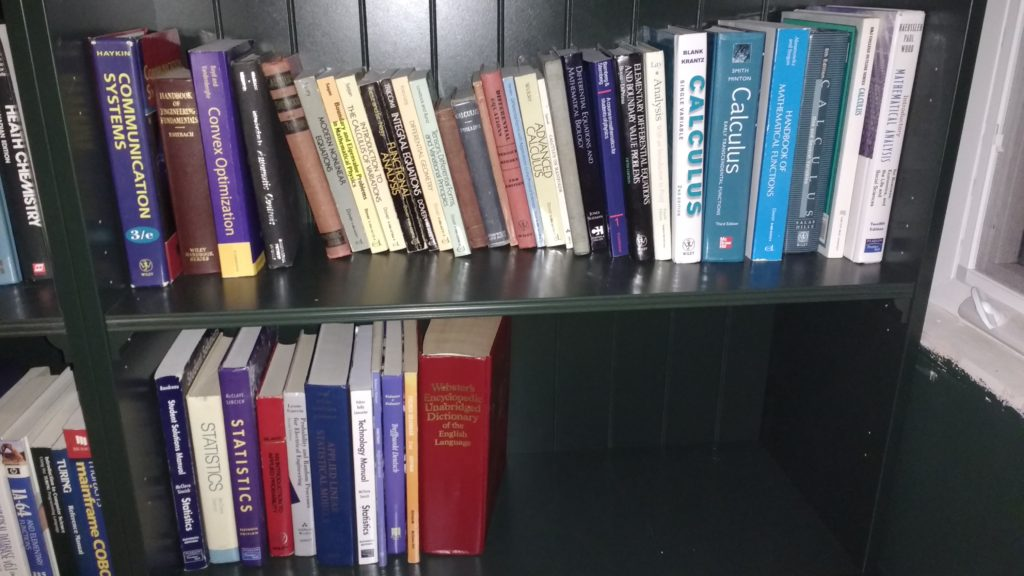• Computer programming, including my brand new Knuth box set!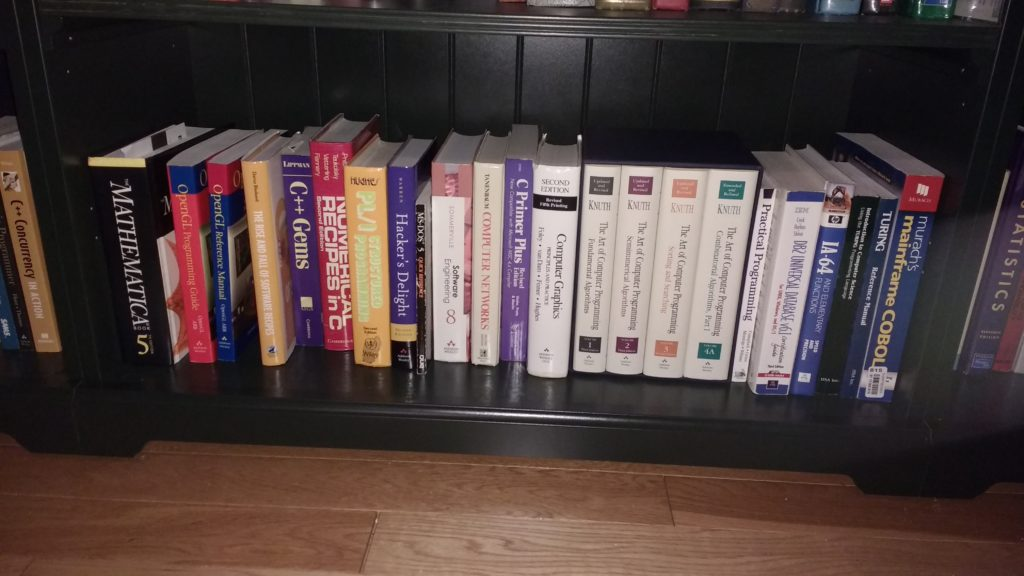•  Spill over programming, general physics, fluid mechanics, and solid mechanics: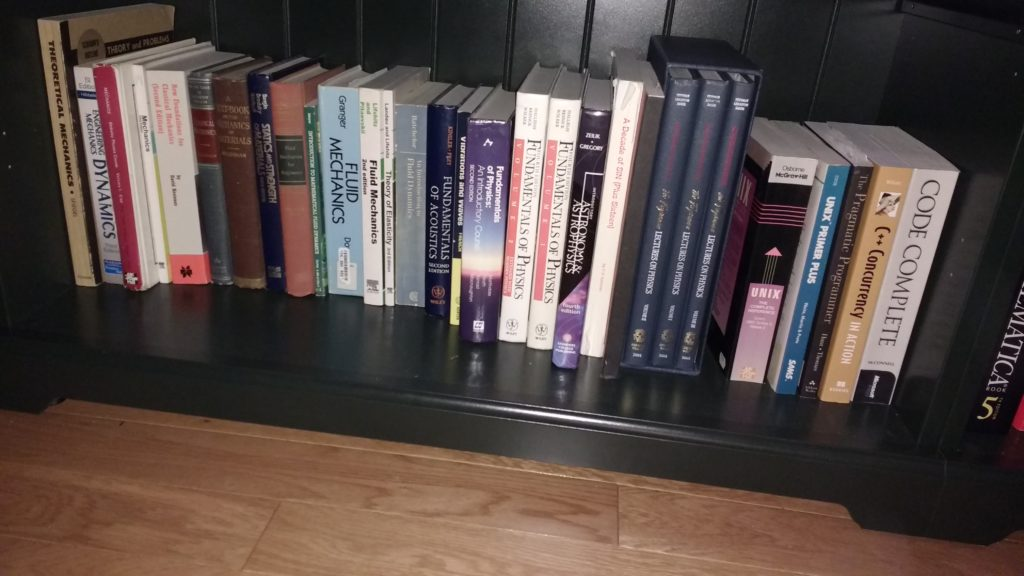• Home repair and handywork, plus two religious books too big to fit in the religion section (I’ve got other religious material in boxes somewhere in the basement, including a Morman bible, a Koran, and a whole lot of Dad’s Scientology books (and a couple of mine from days of old) :• Optics and statistical mechanics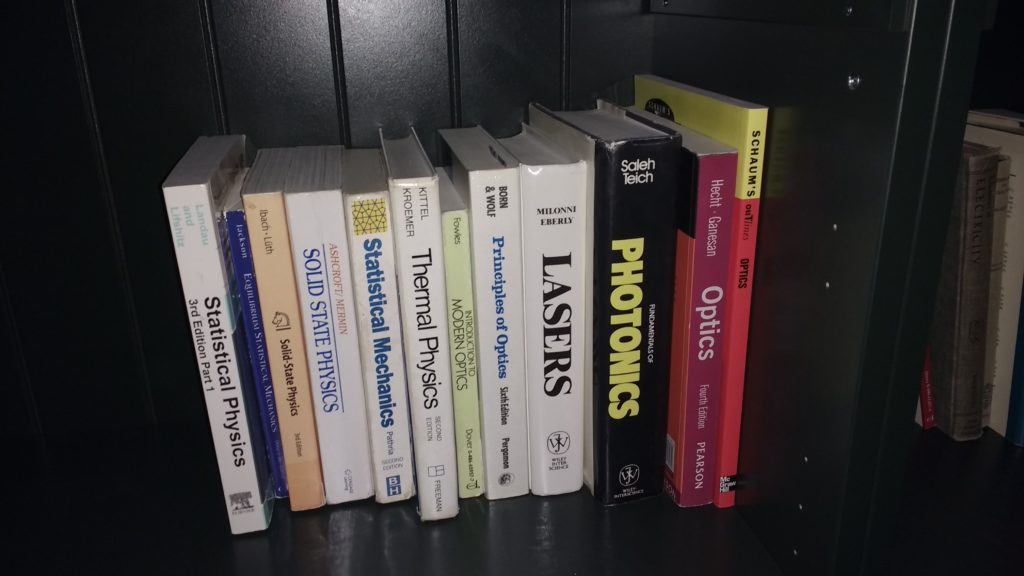• Investment and economics (although the only one I’ve really cracked of these is the old “Principles of Engineering Economic Analysis” from back in my undergrad days)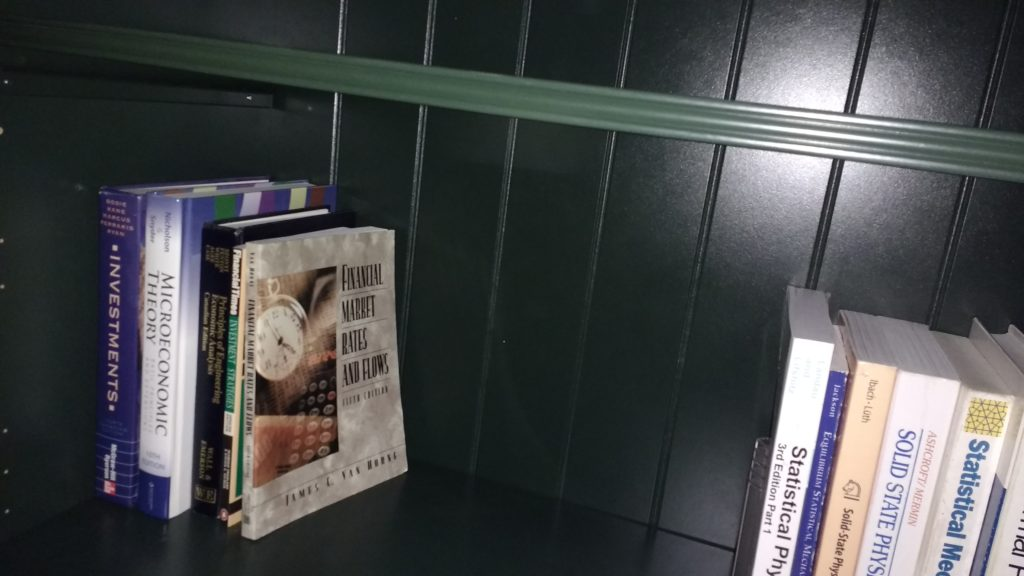• Electromagnetism and some older general physics books from Granddad: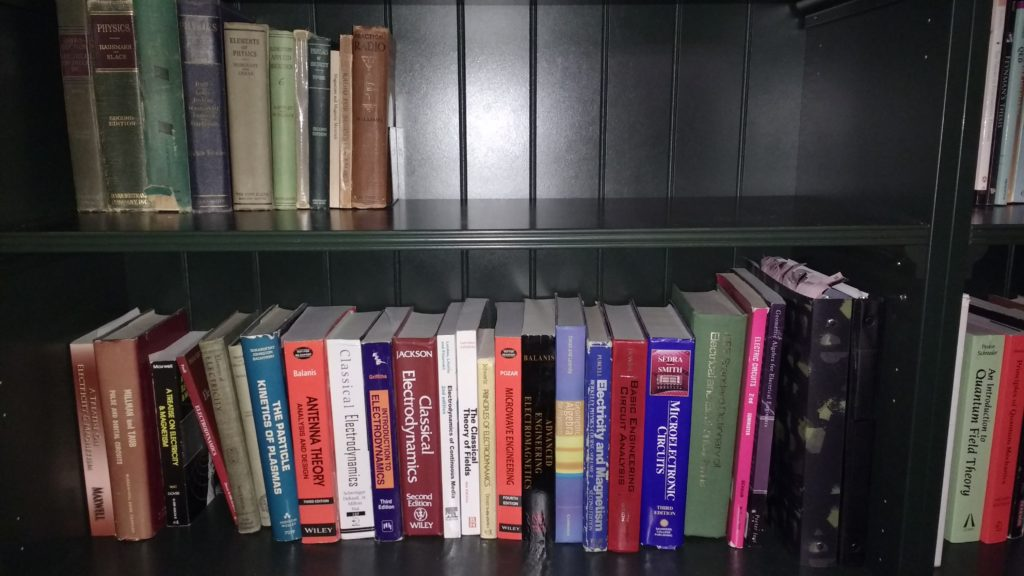• Algebra, complex variables, General relativity, mathematical tables, plus Penrose’s book, which spans most categories: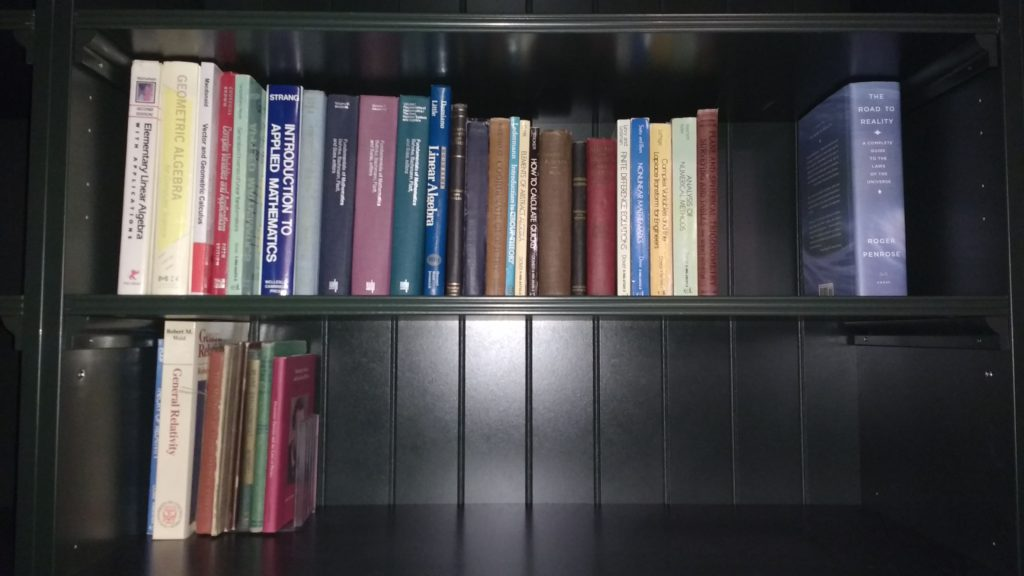• Political, classics, some borrowed Gaiman books, and religious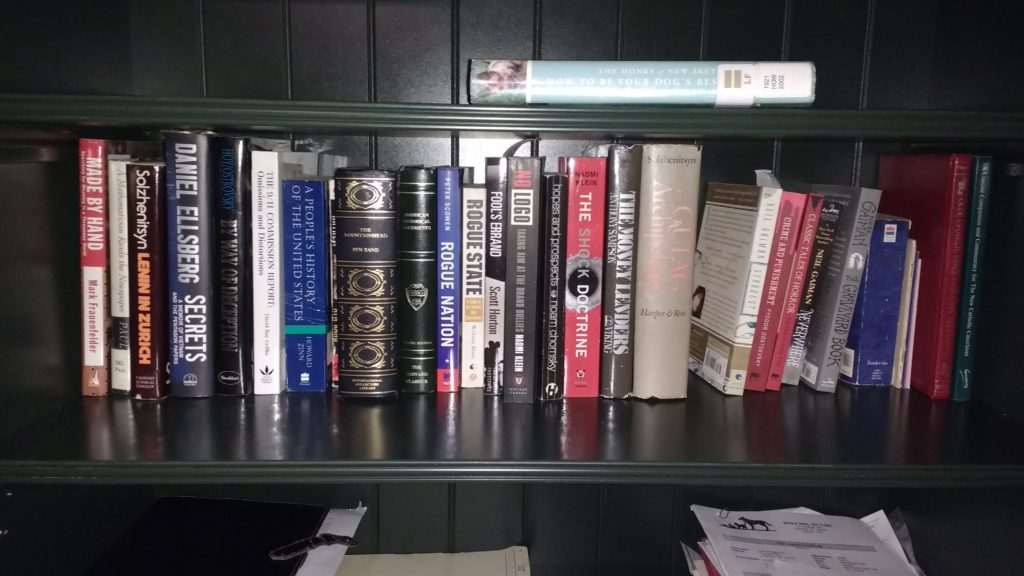• Languages: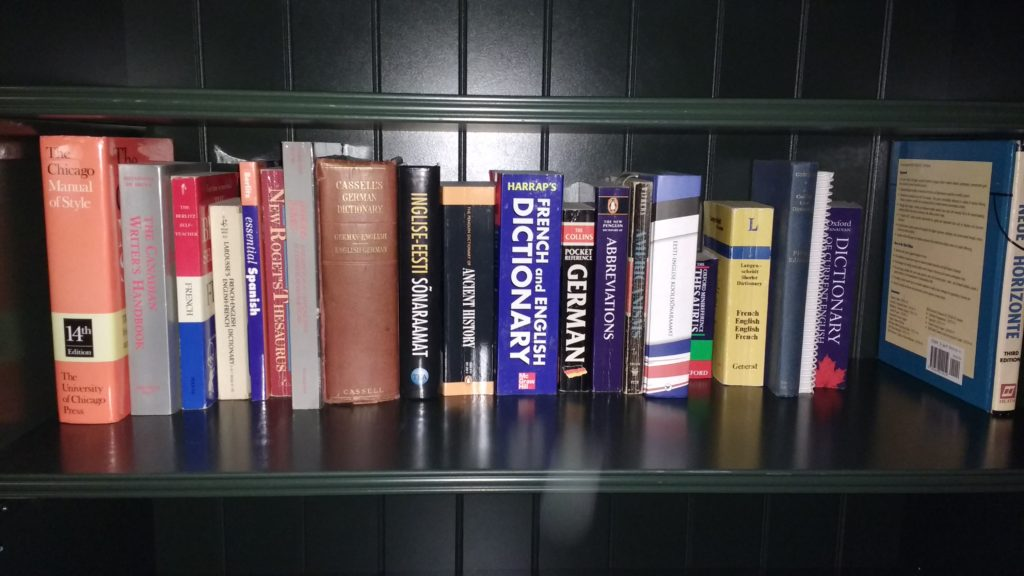There’s a bunch of tidy up and finishing details to make my office space complete and usable, but this was a really nice step in that direction.  Mysteriously, even after moving all these books downstairs from the bedroom, somehow the bedroom bookshelves are still mostly full seeming.  Was there a wild book orgy when we weren’t looking, and now all the book progeny are left behind, still filling the shelves despite the attempt to empty them?